# RS Aggarwal Solutions for Class 9 Maths Chapter 7: Lines and Angles

Angles are formed when two rays start from the same point. The rays forming an angle are known as arm and the end point is the vertex of the angle. There are different kinds of angles namely reflex angle, obtuse angle, right angle, complete angle, straight angle and acute angle. The different types of lines mainly include skew lines, vertical lines, parallel lines, horizontal lines and perpendicular lines.

RS Aggarwal Solutions for Class 9 helps the students to score good marks in the board exams. The problems are solved accurately with step by step solutions according to the RS Aggarwal Maths text book. RS Aggarwal Solutions for Class 9 Chapter 7 Lines and Angles are provided here.

## RS Aggarwal Solutions for Class 9 Maths Chapter 7 : Lines and Angles Download PDF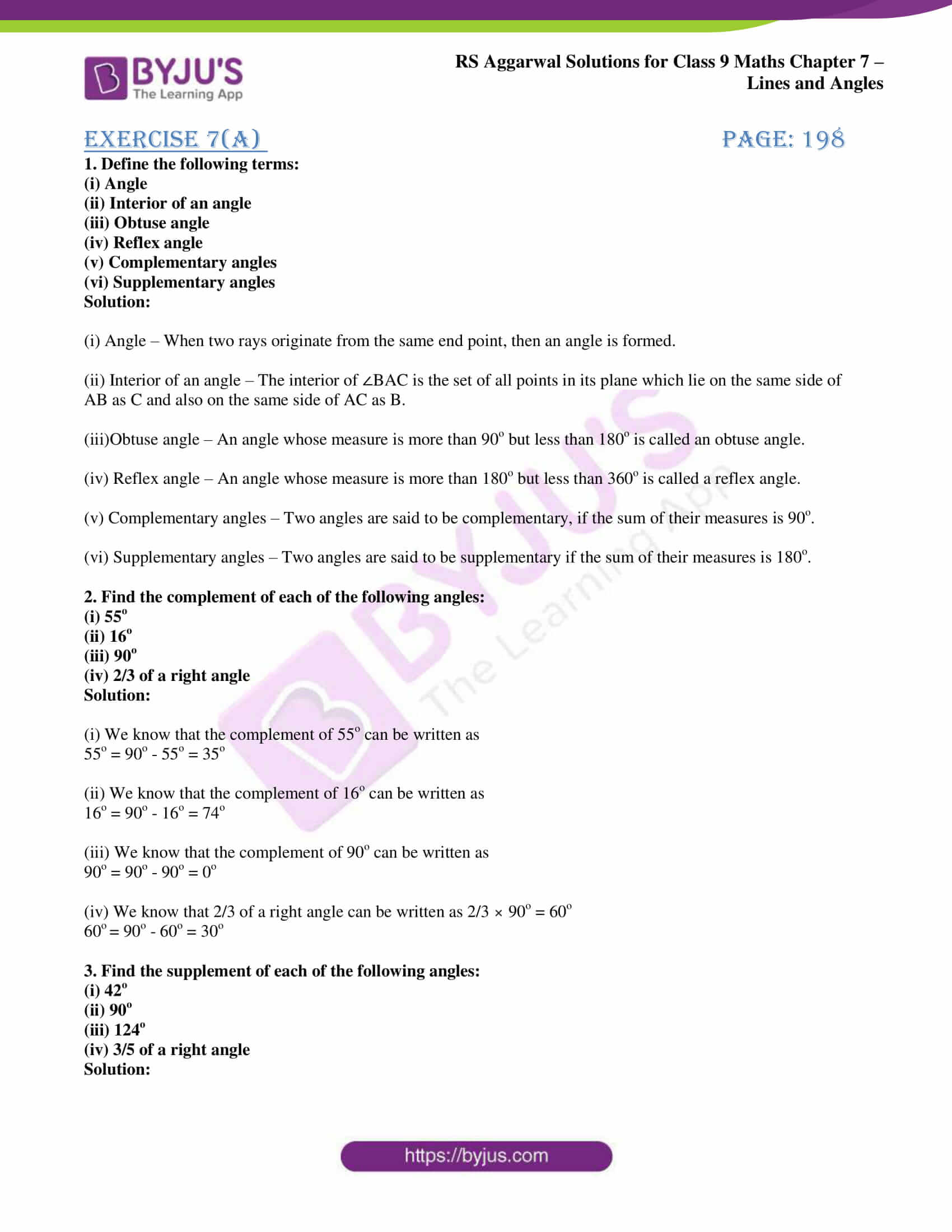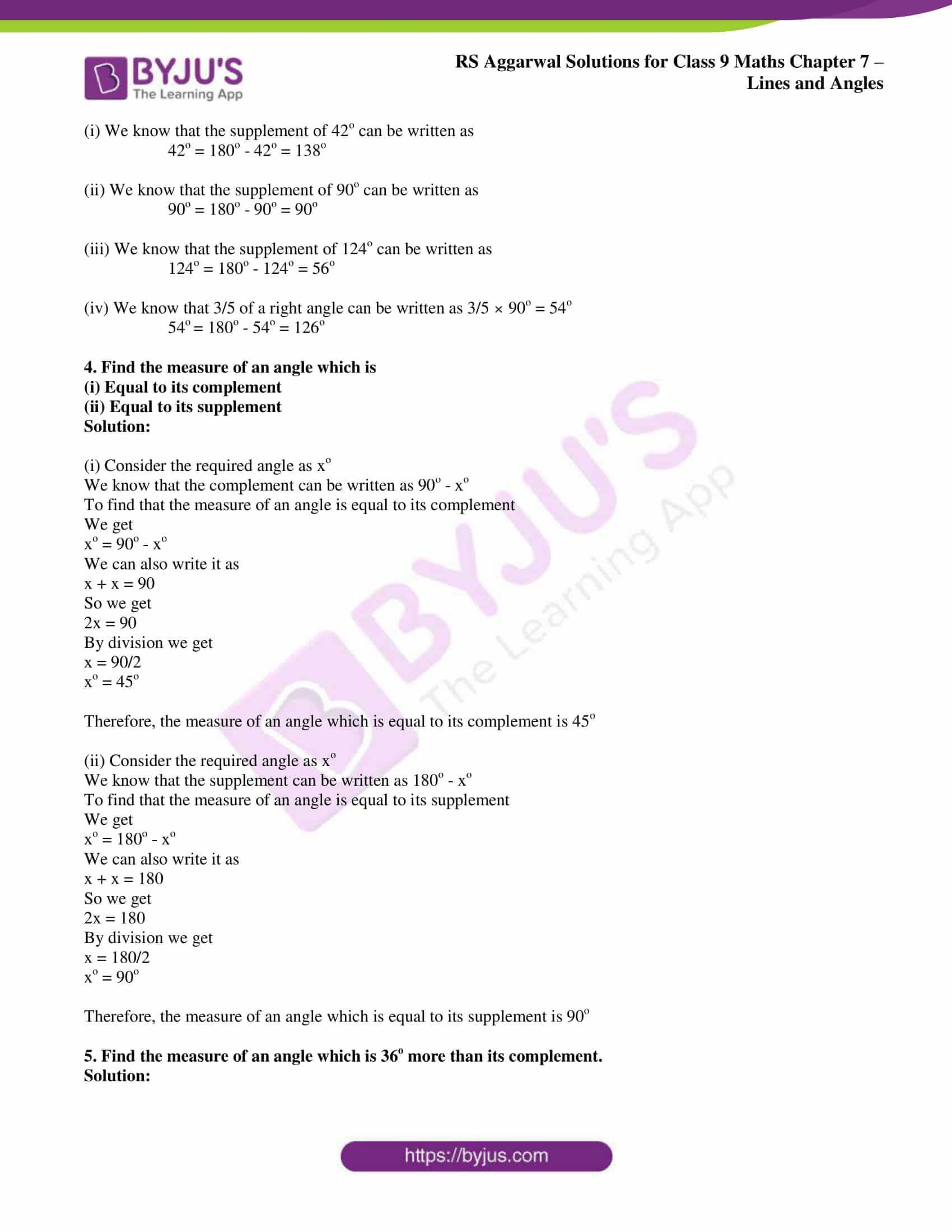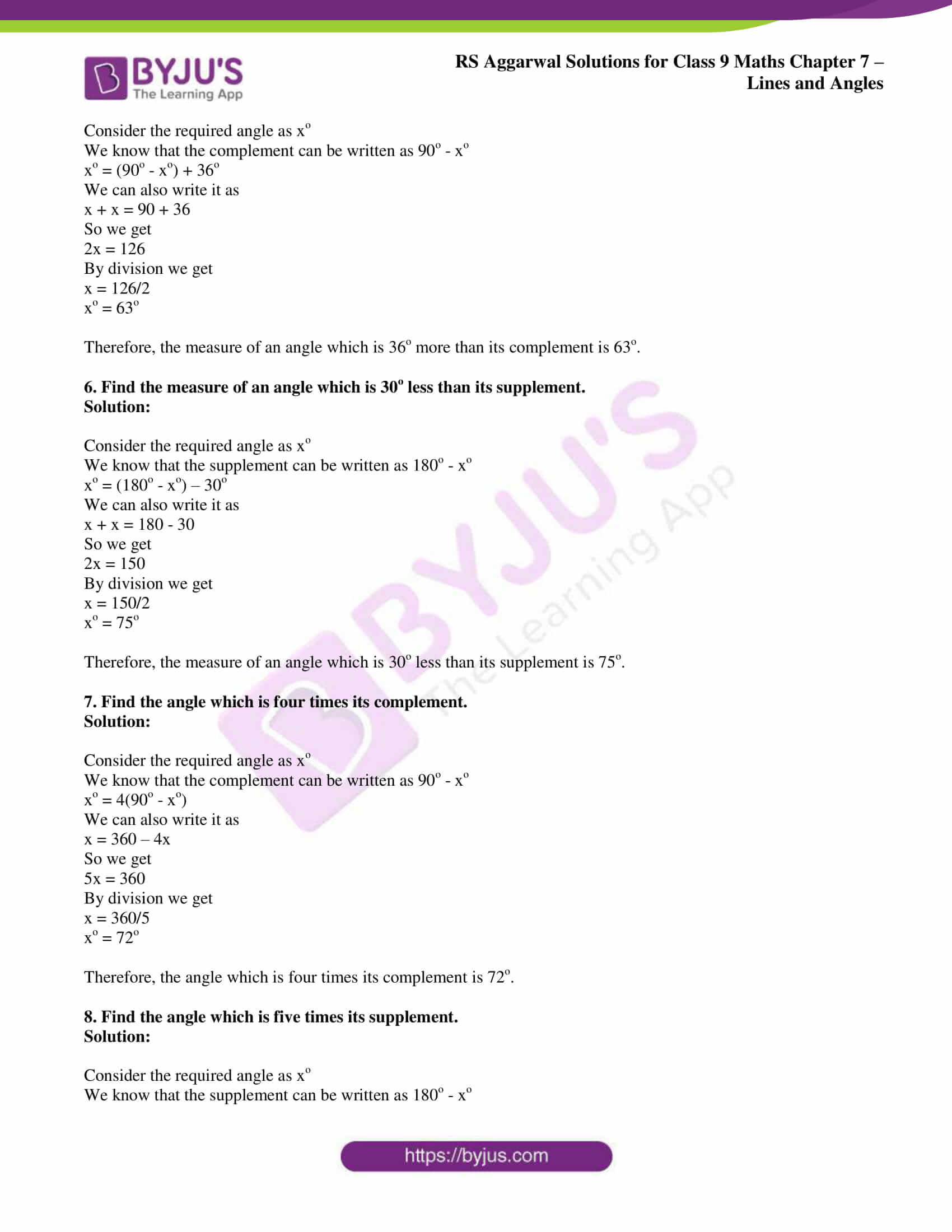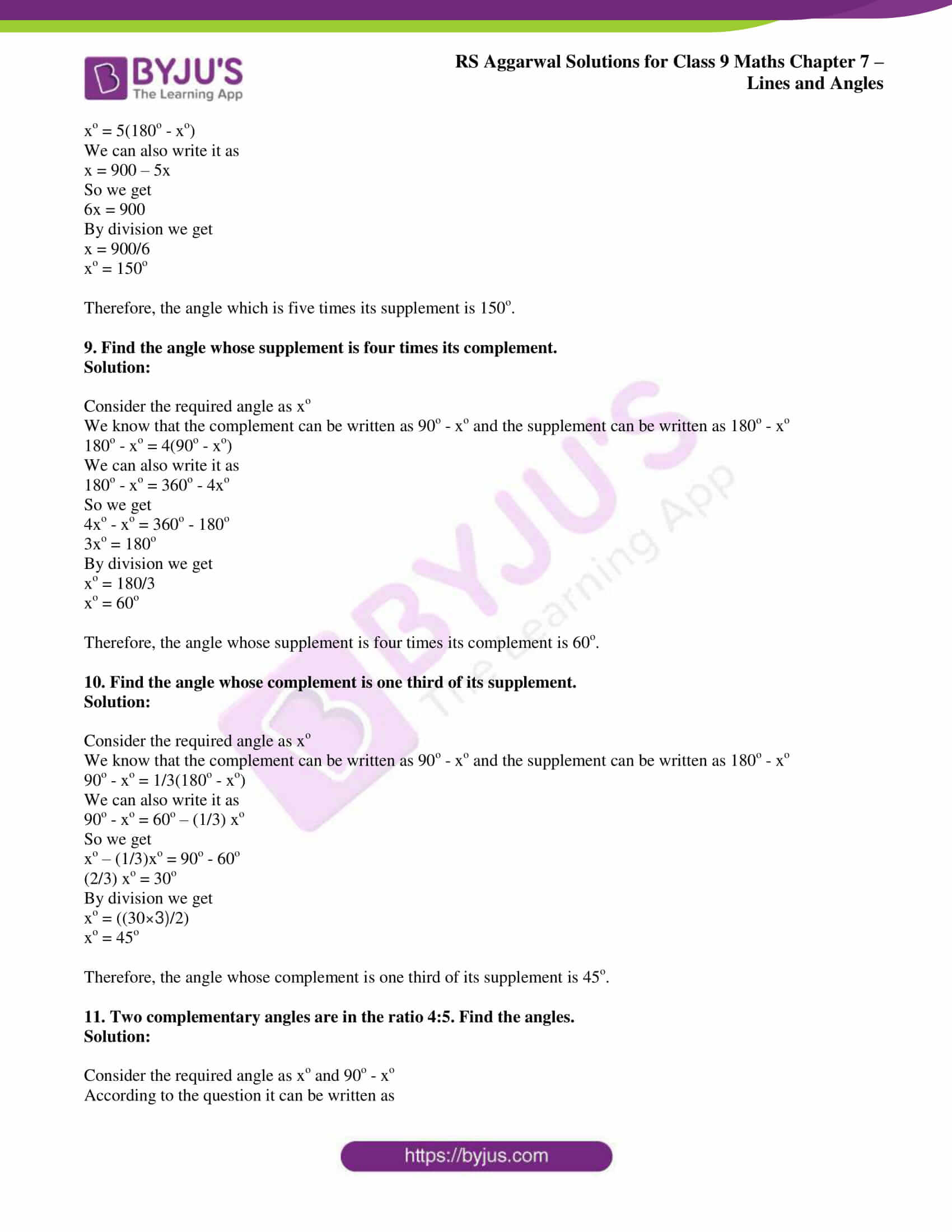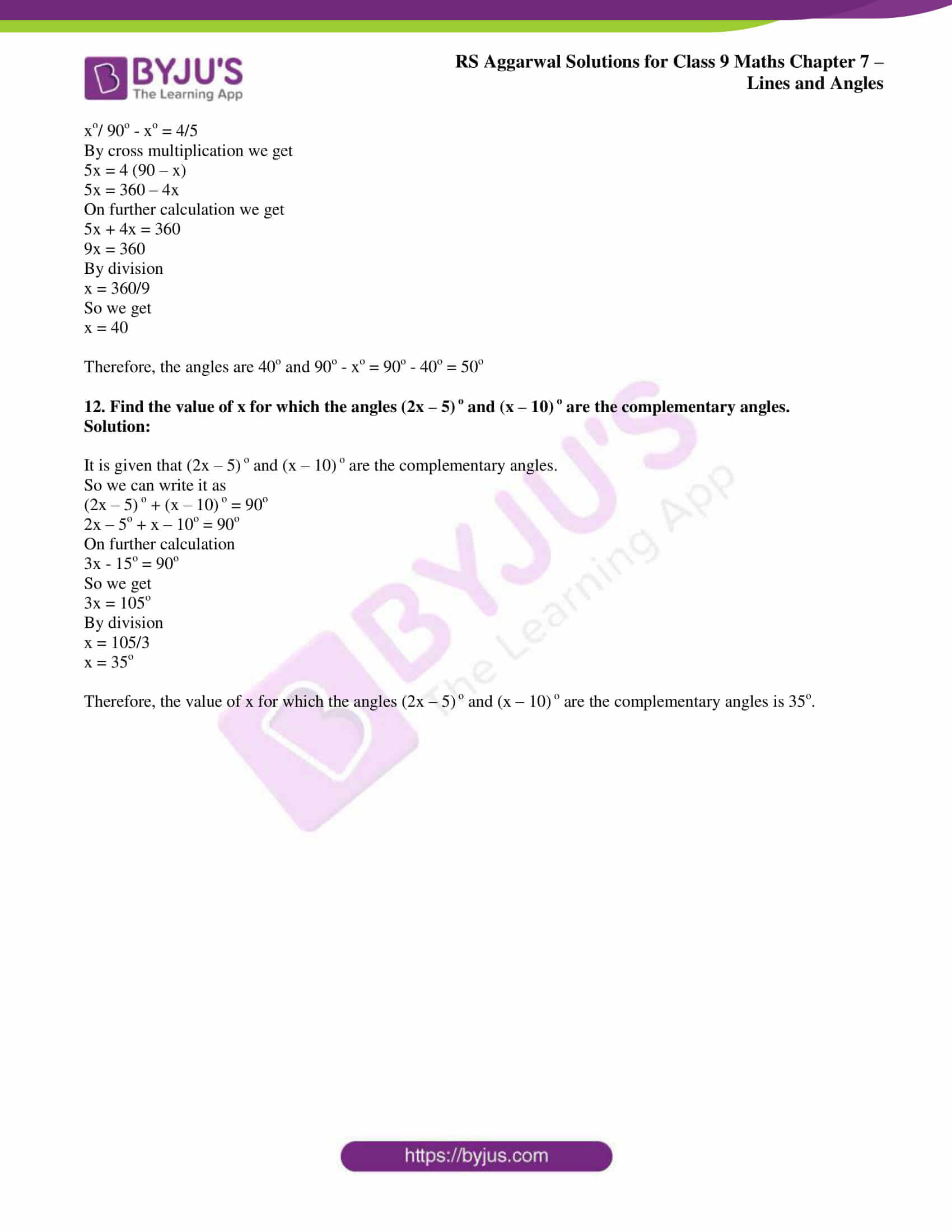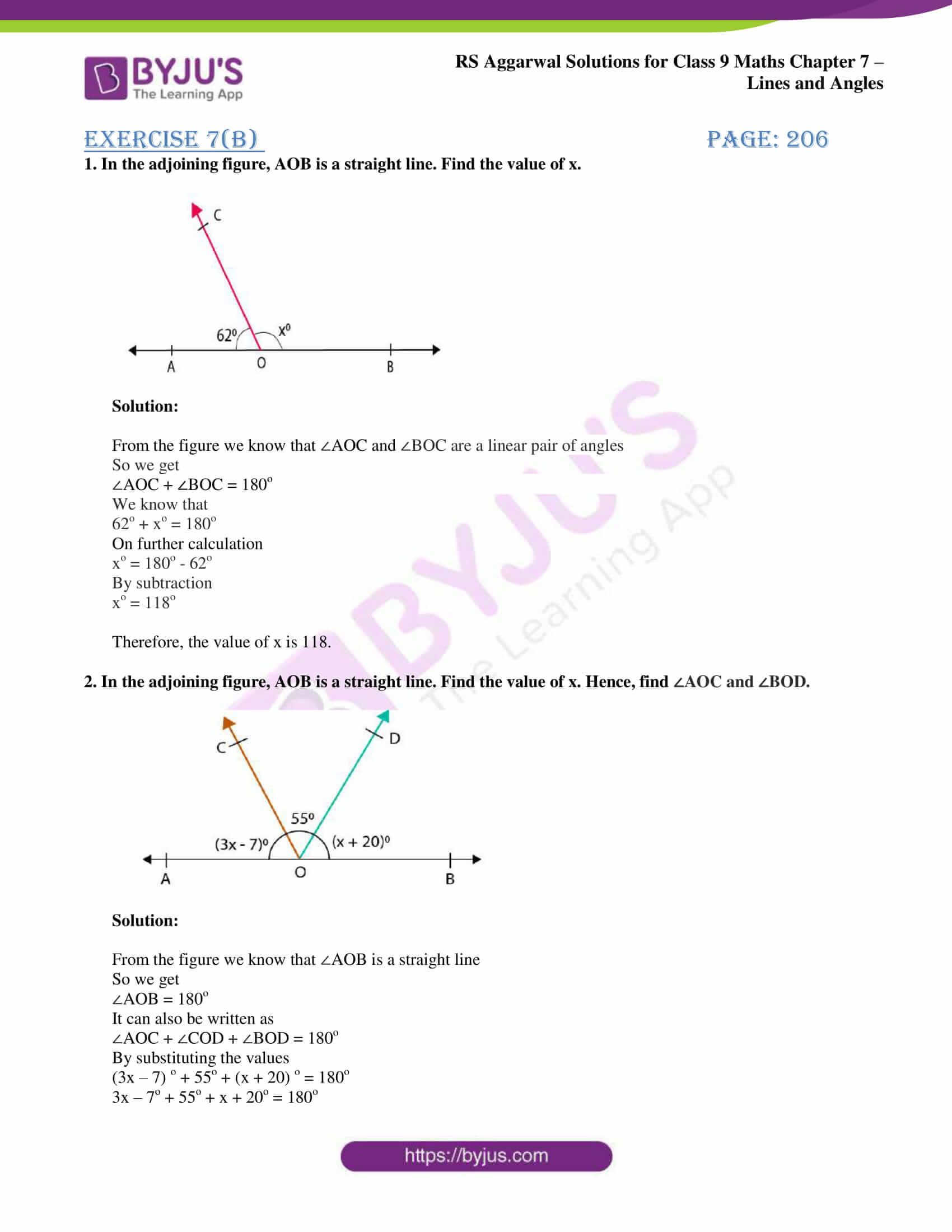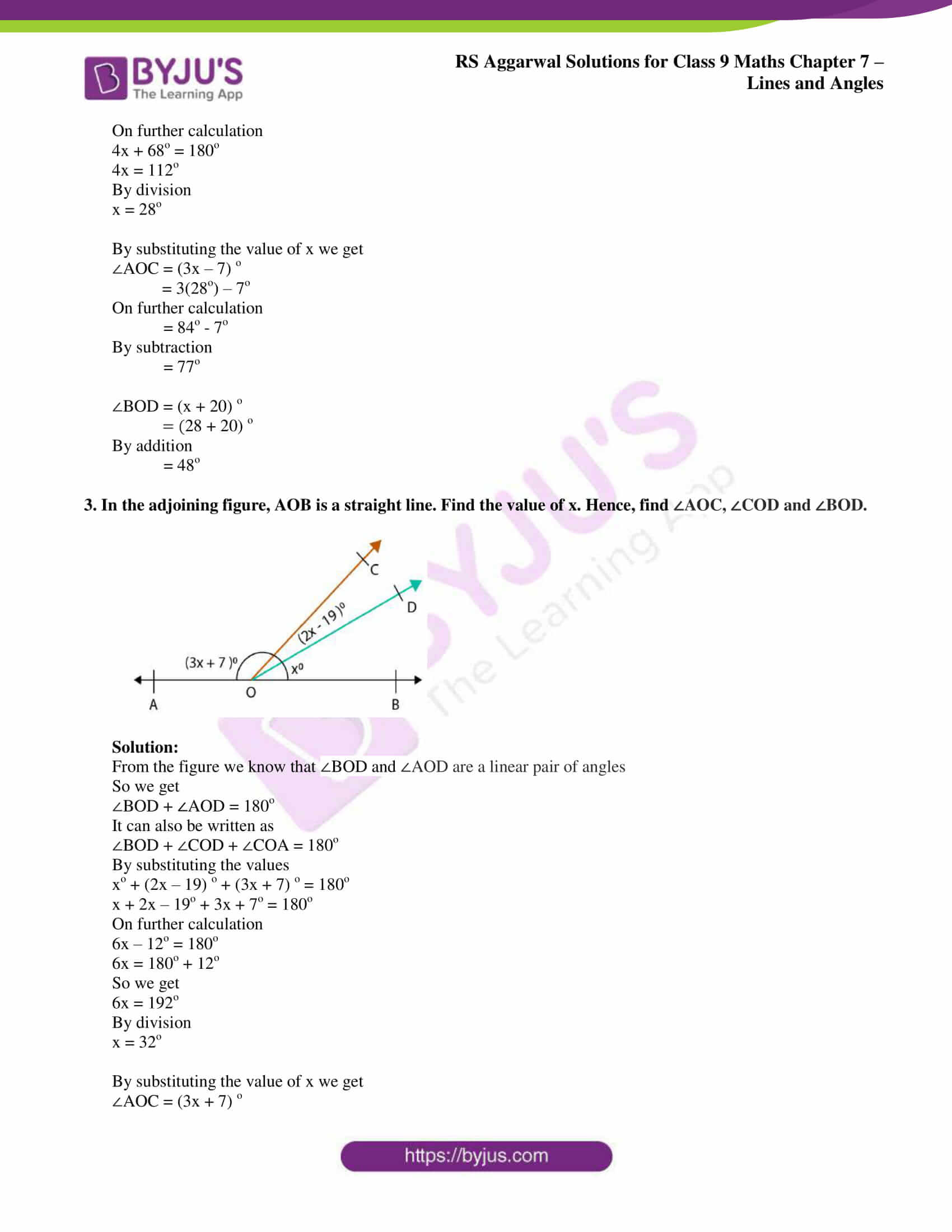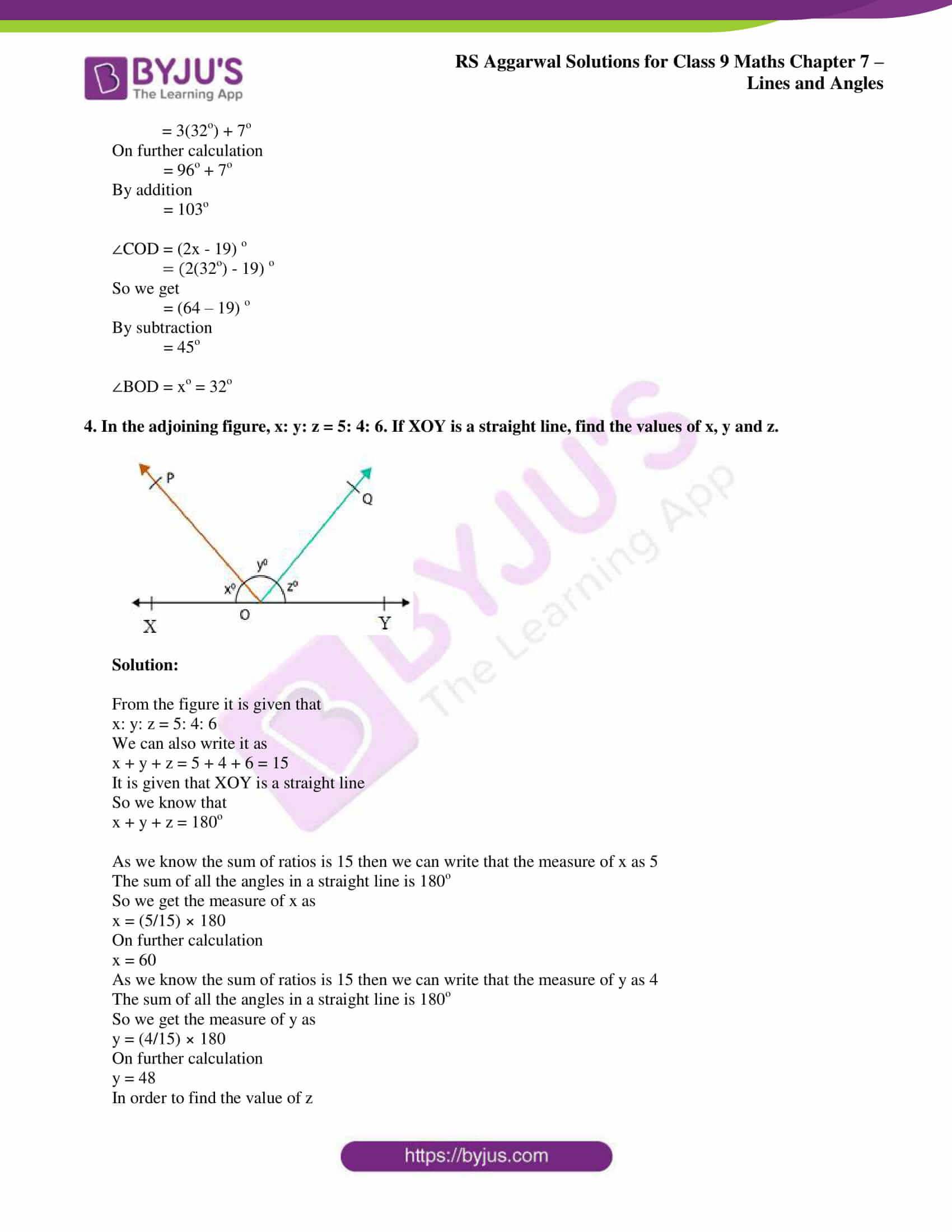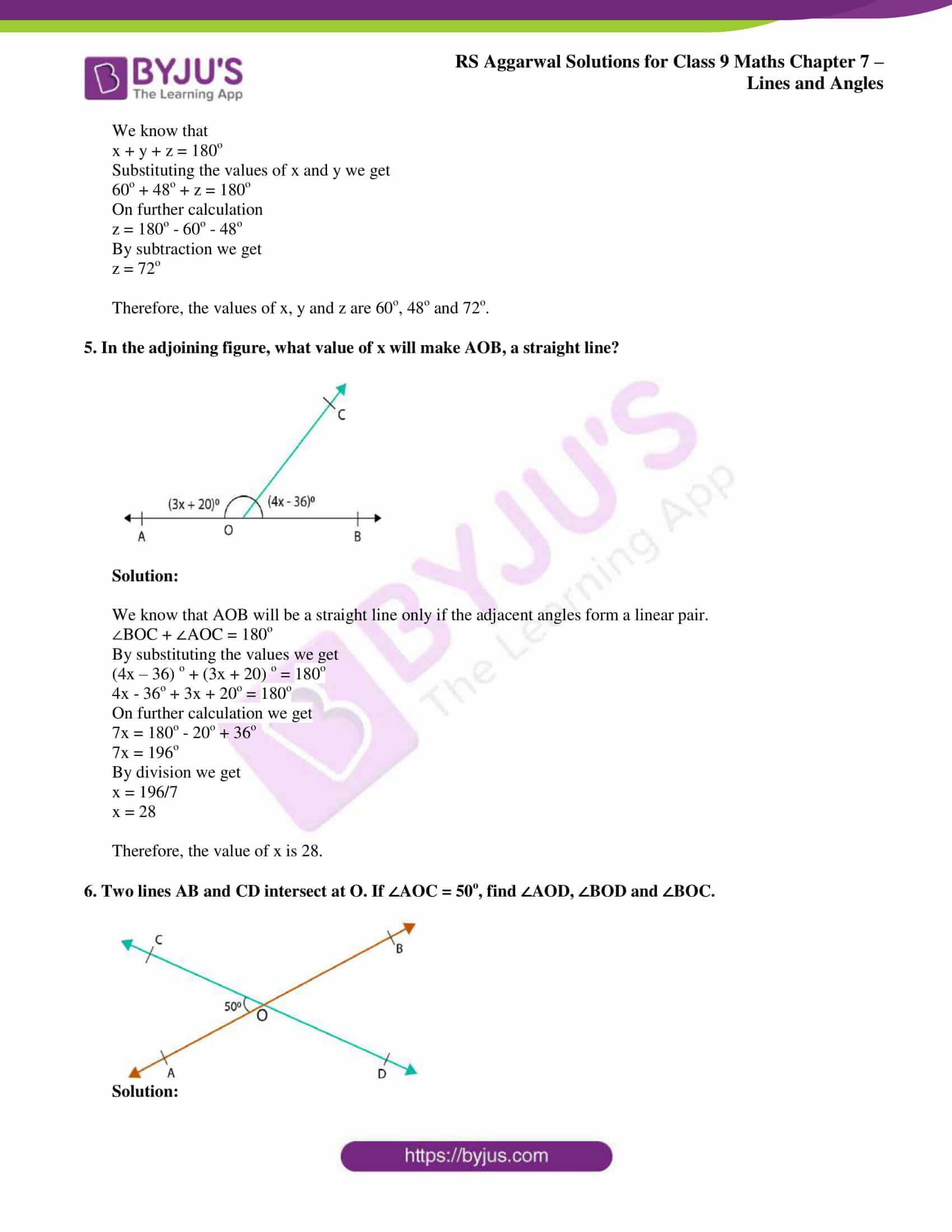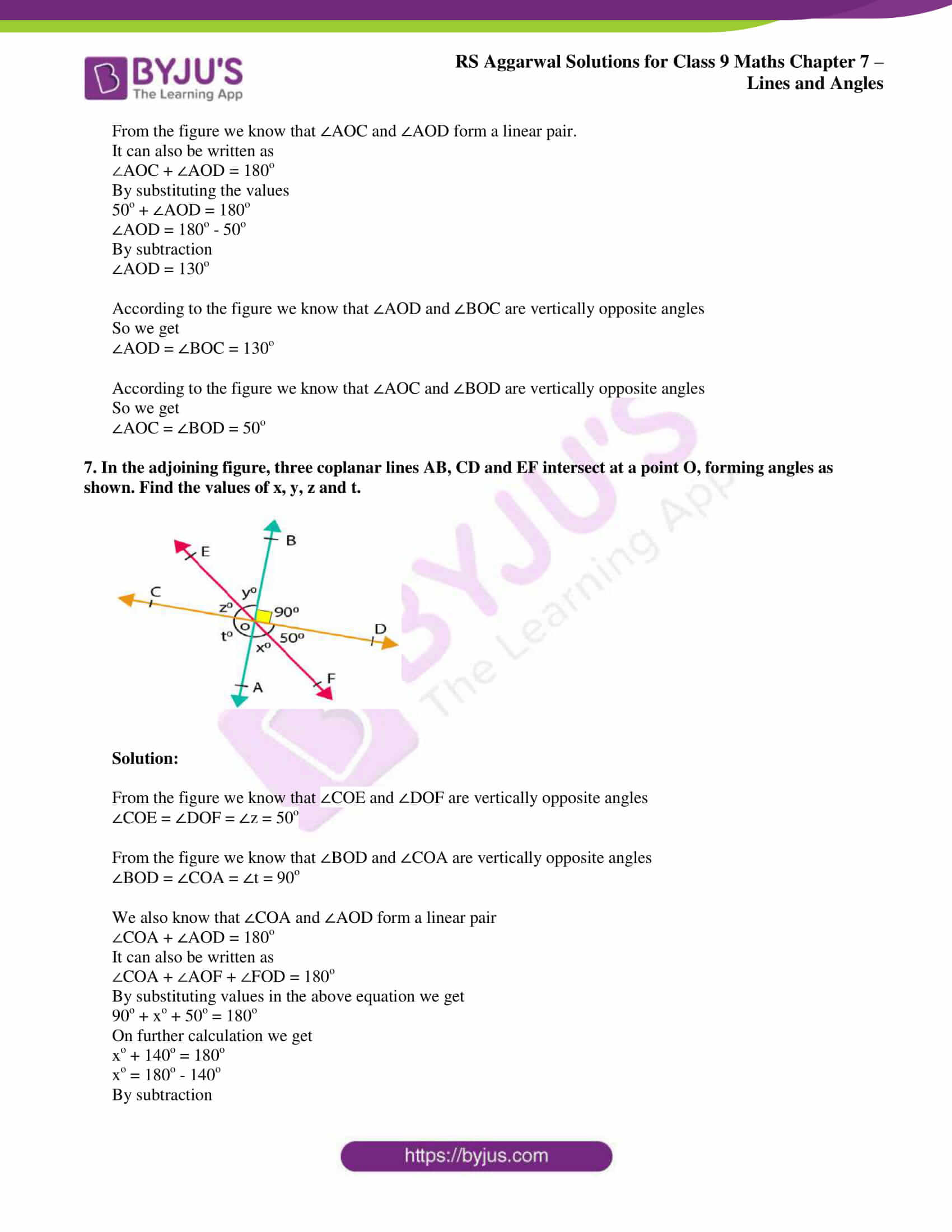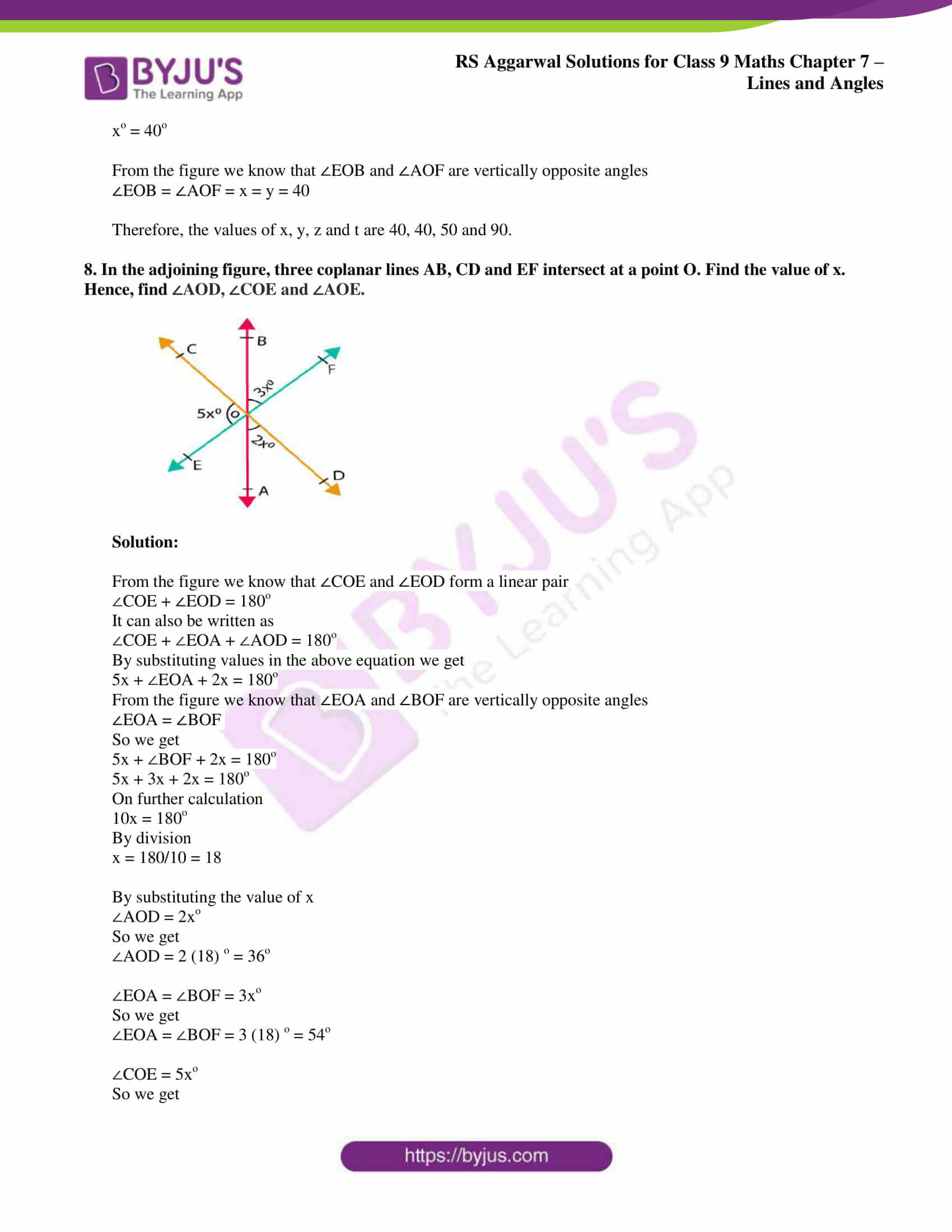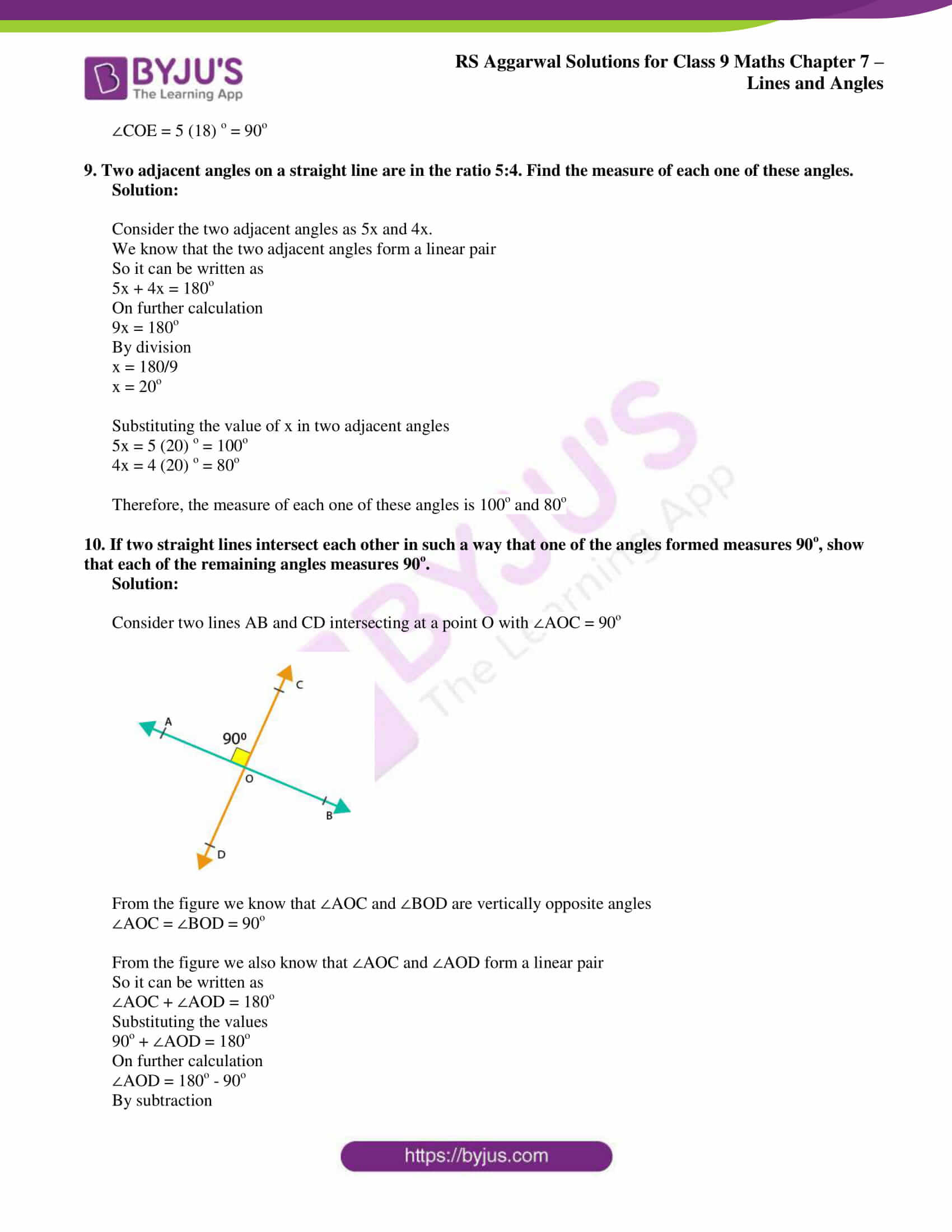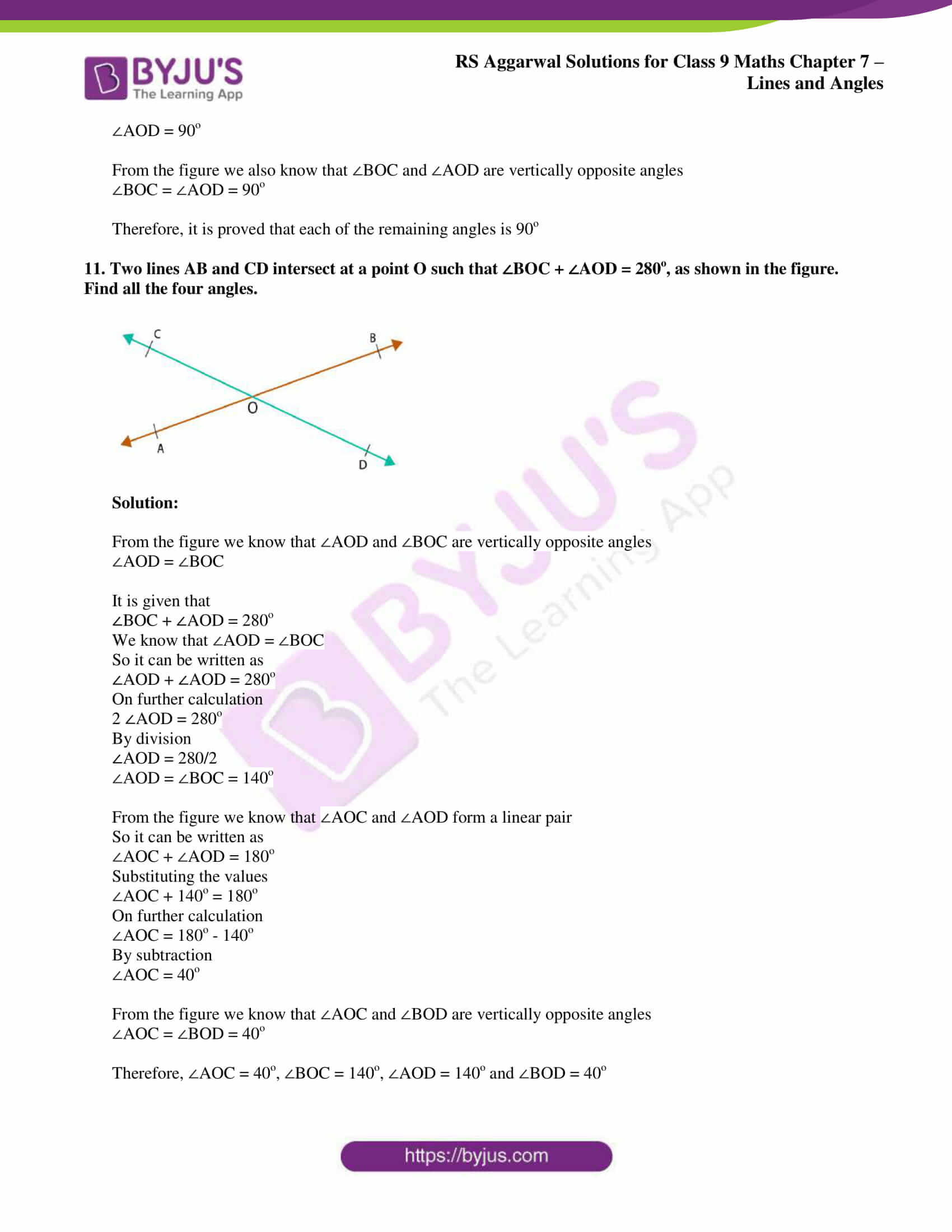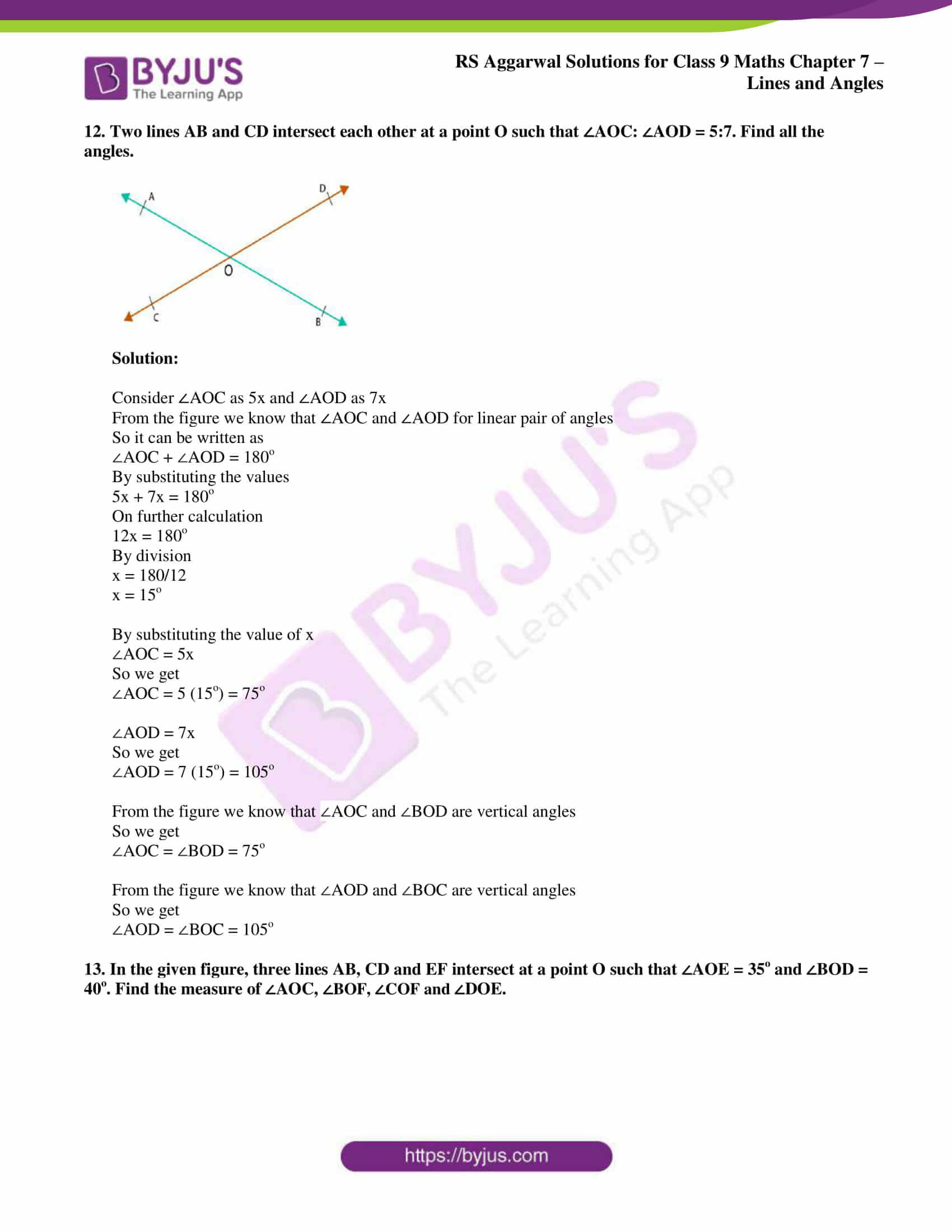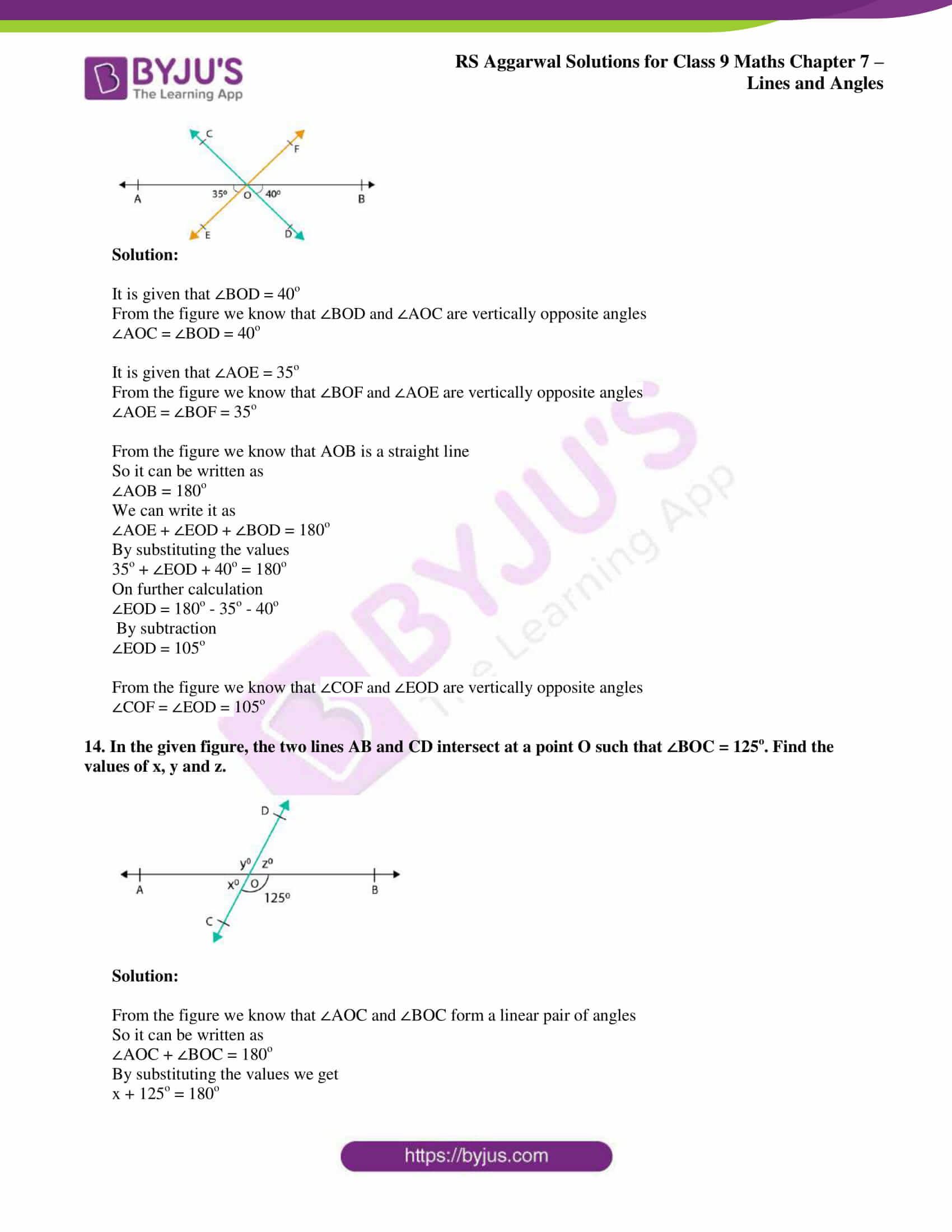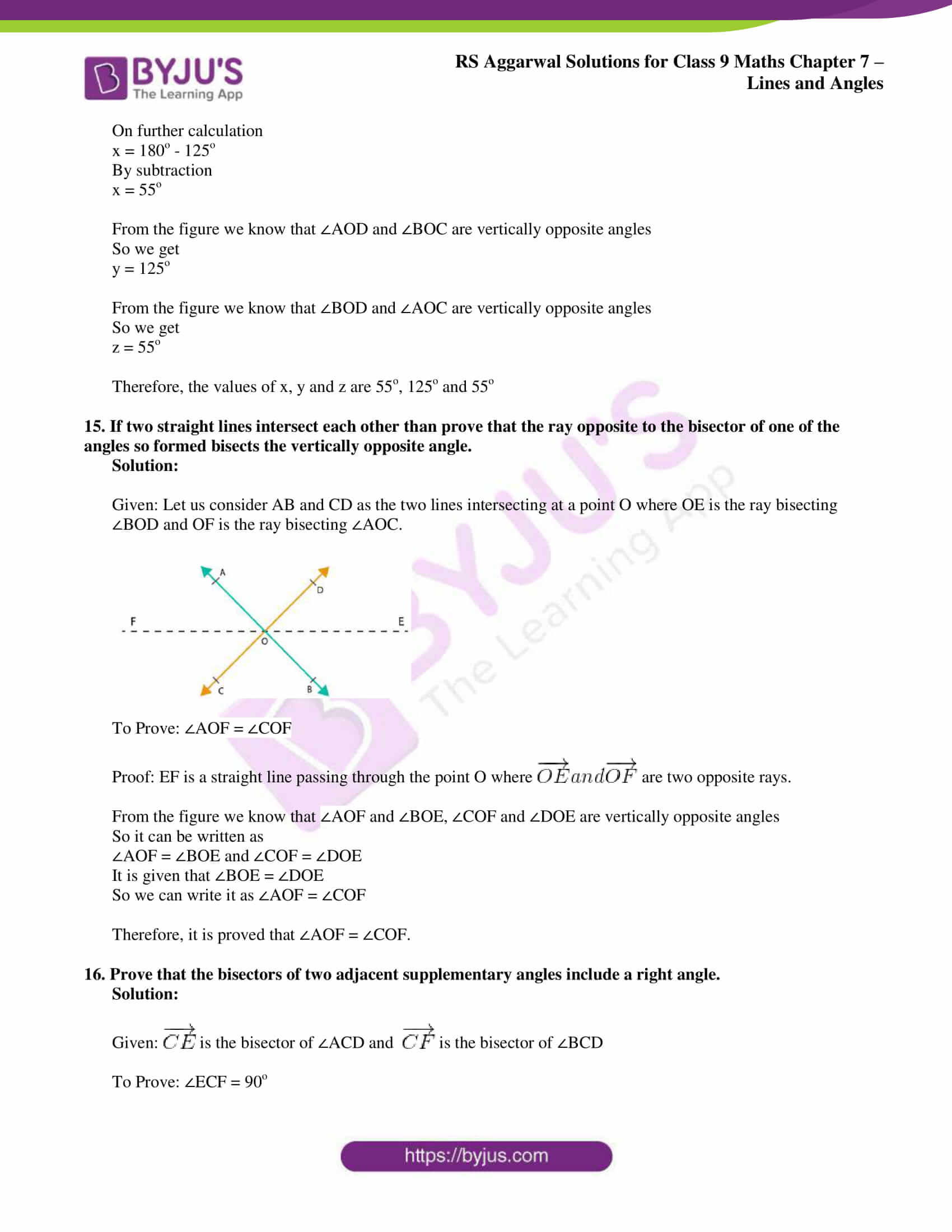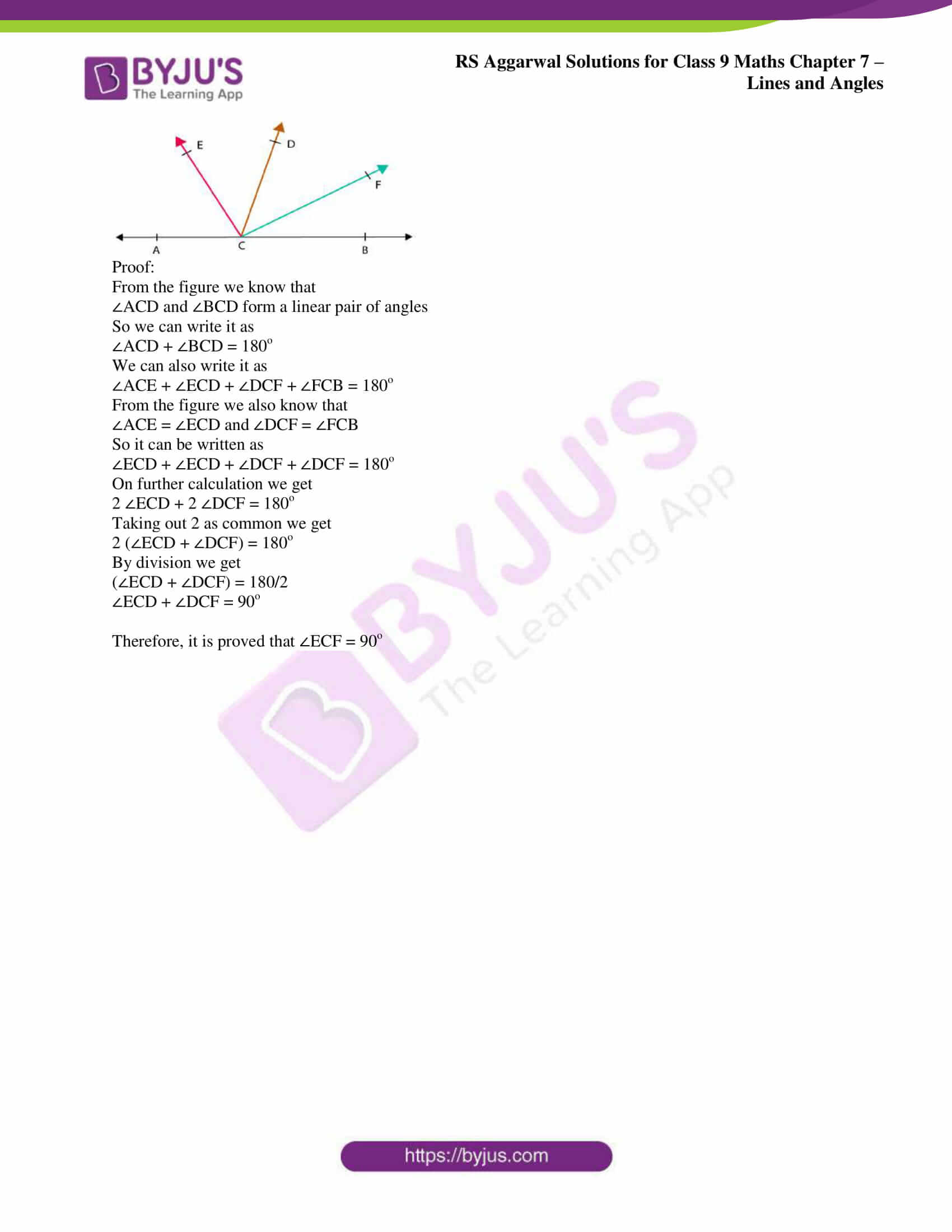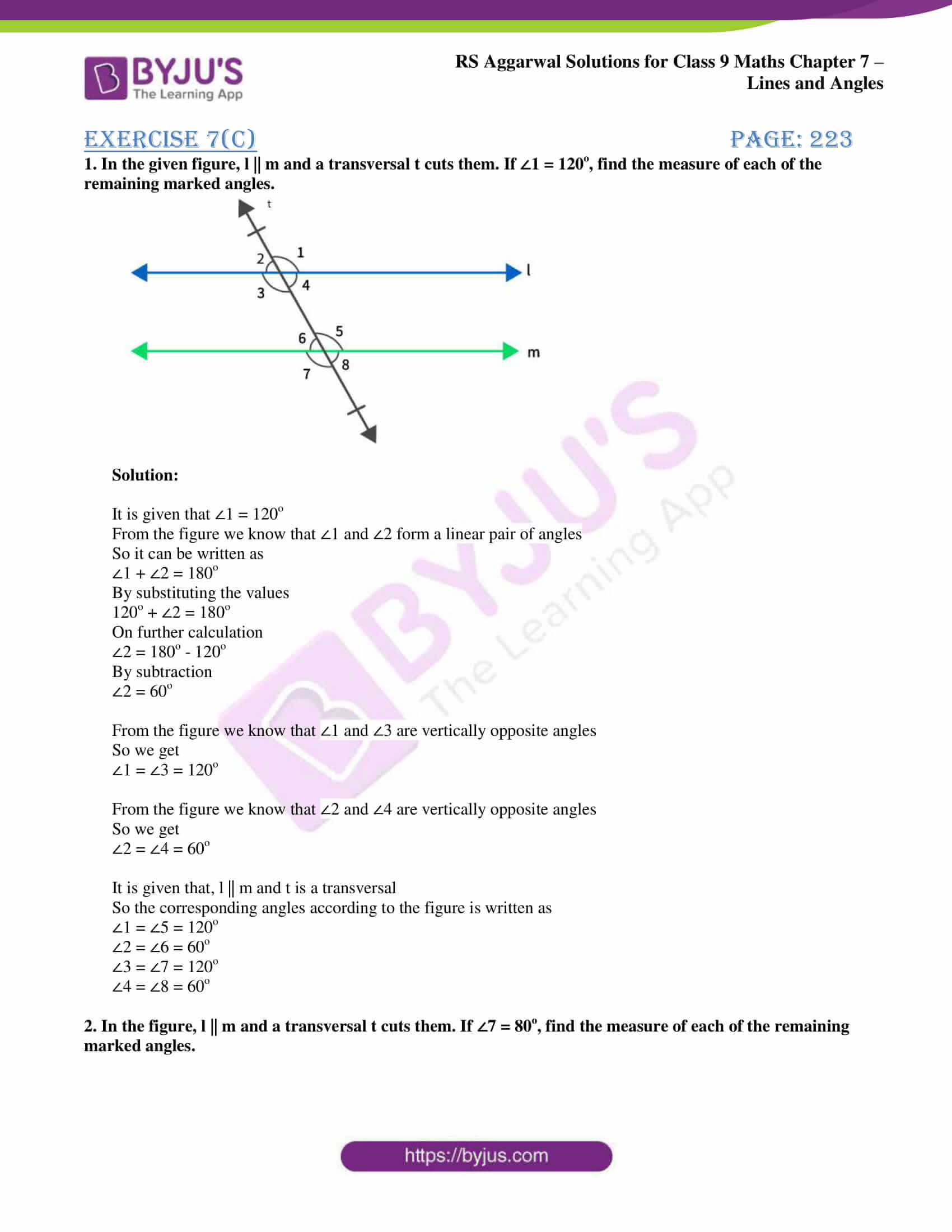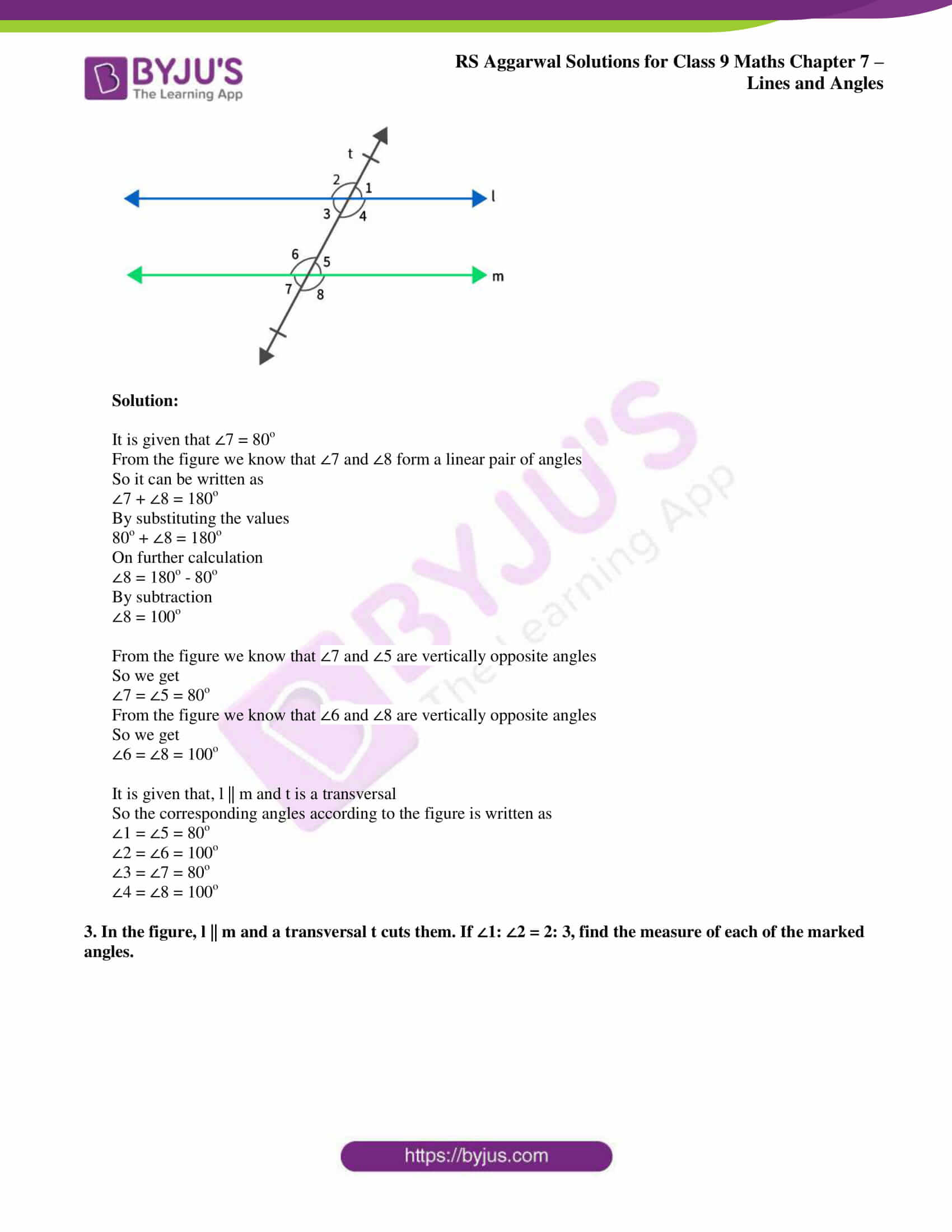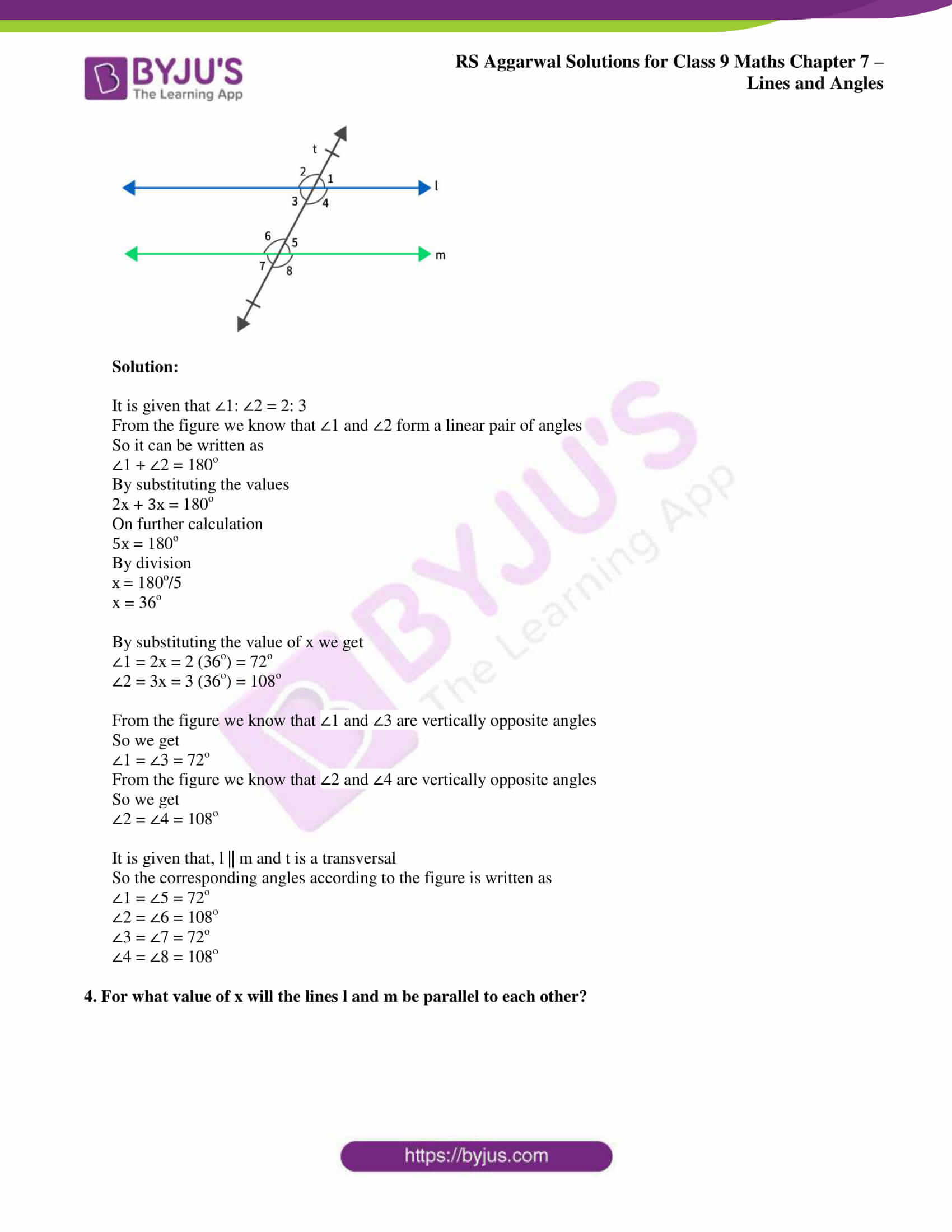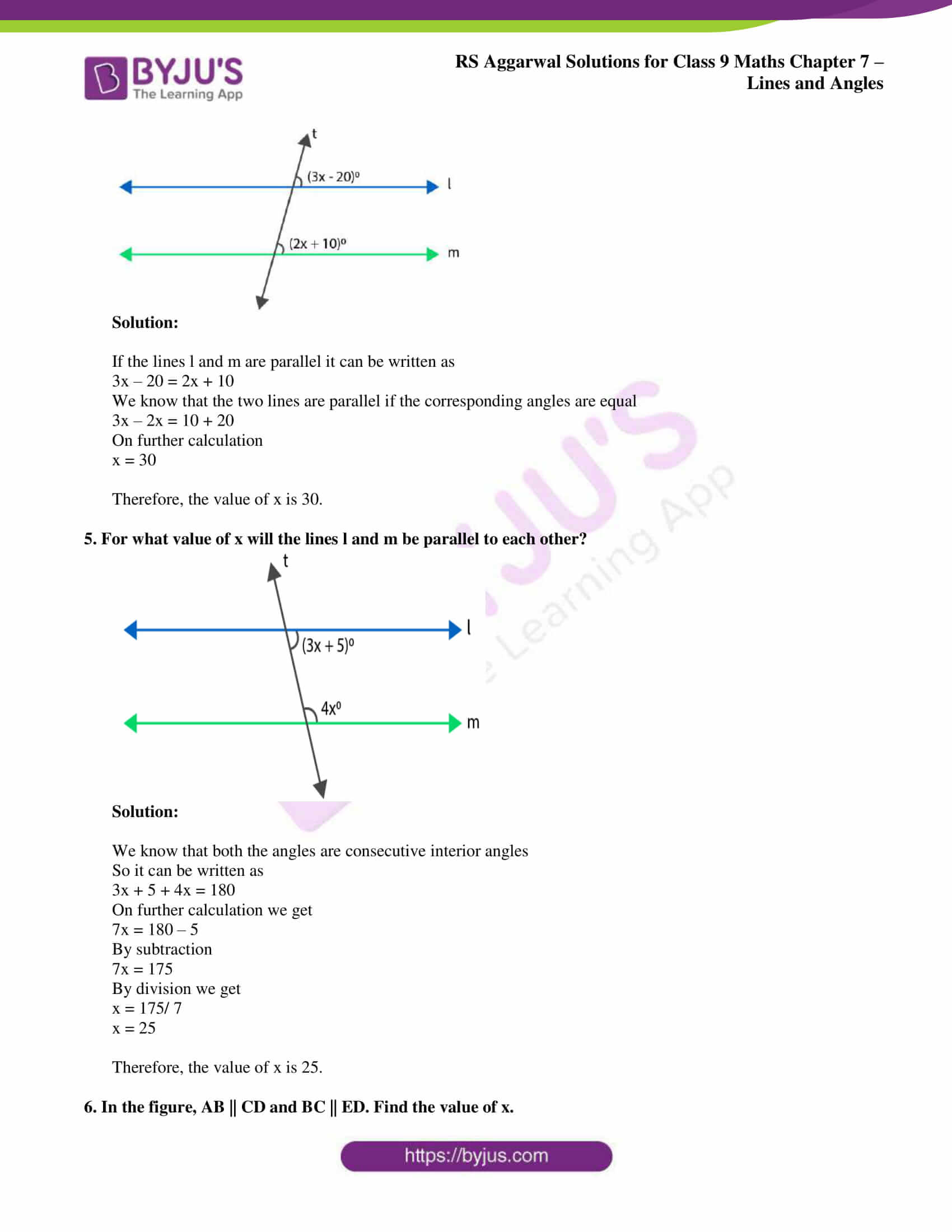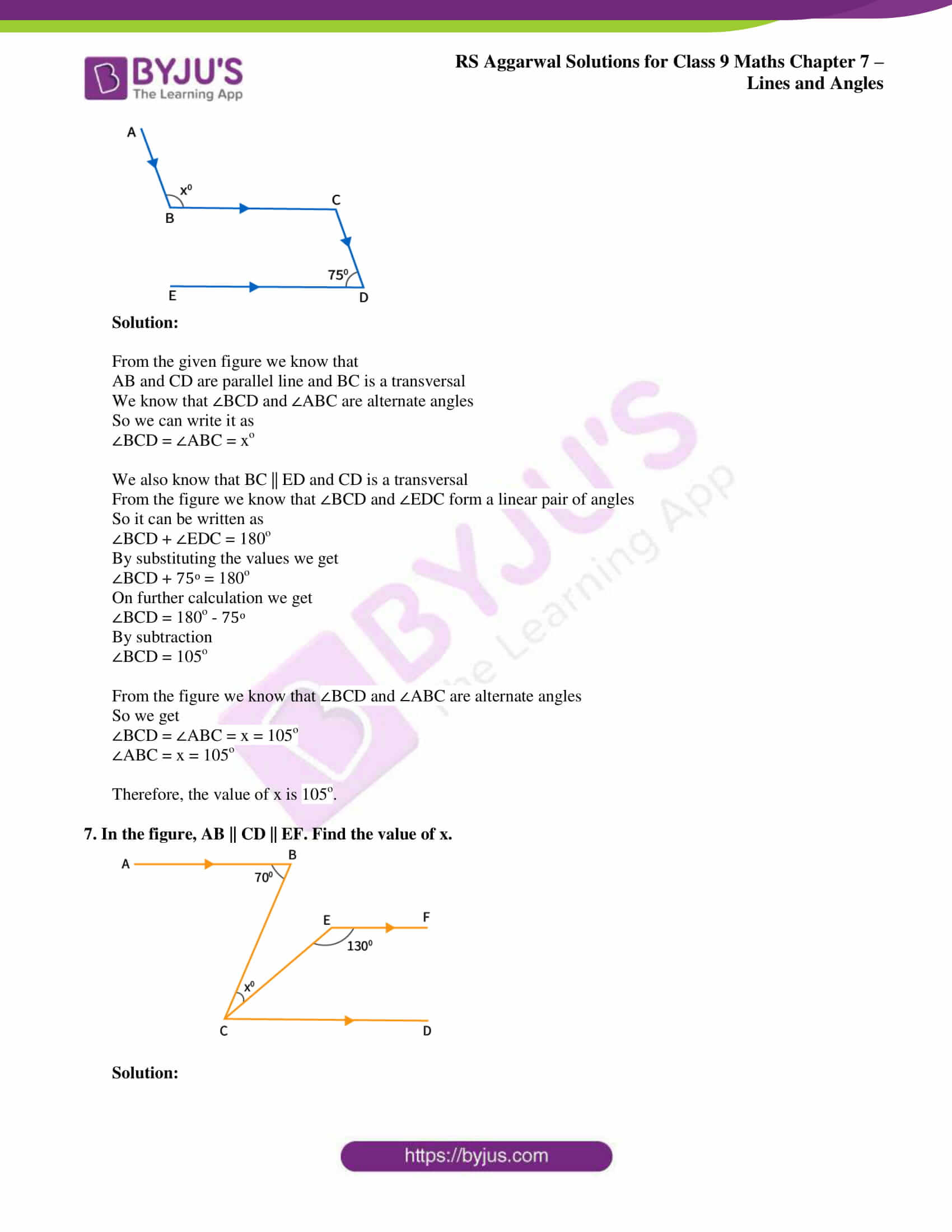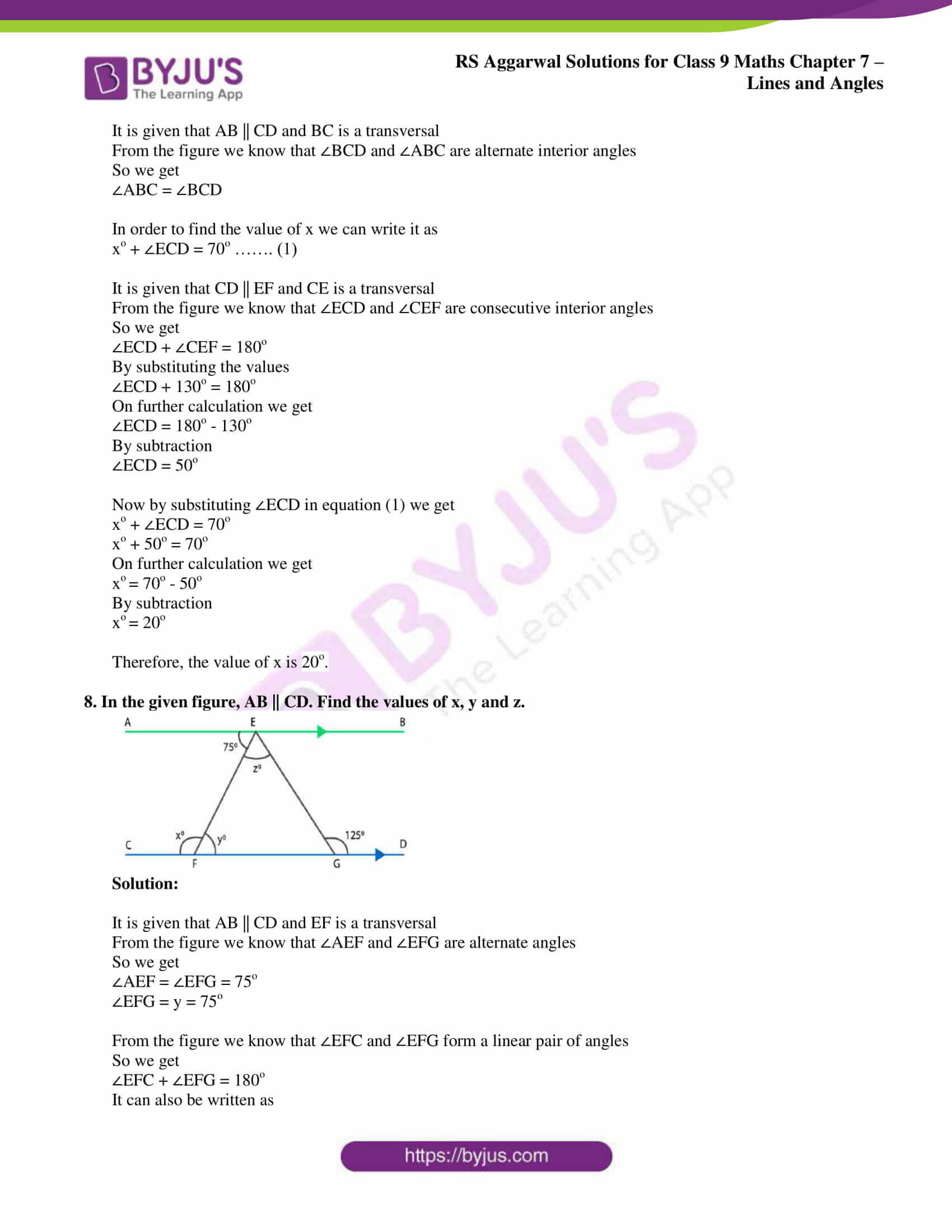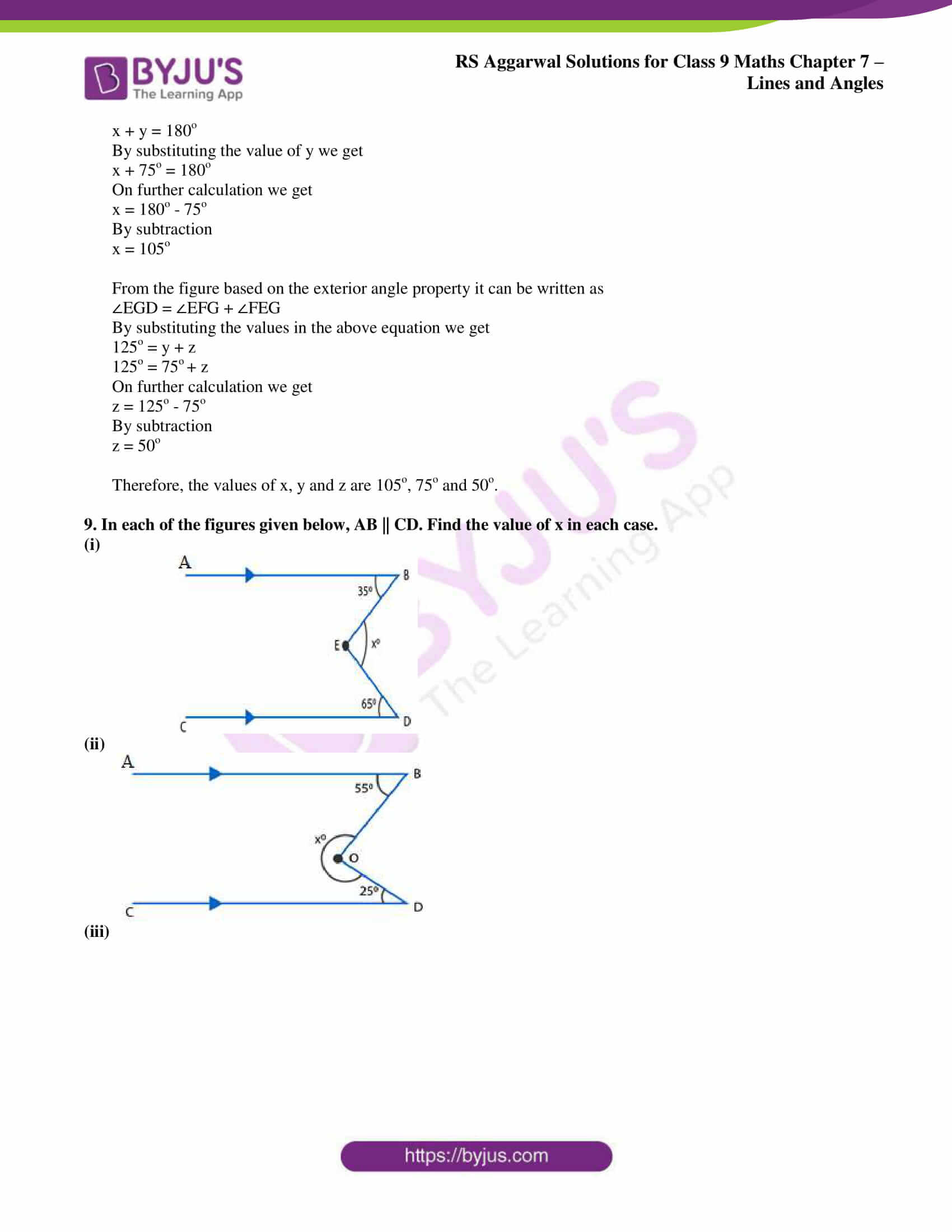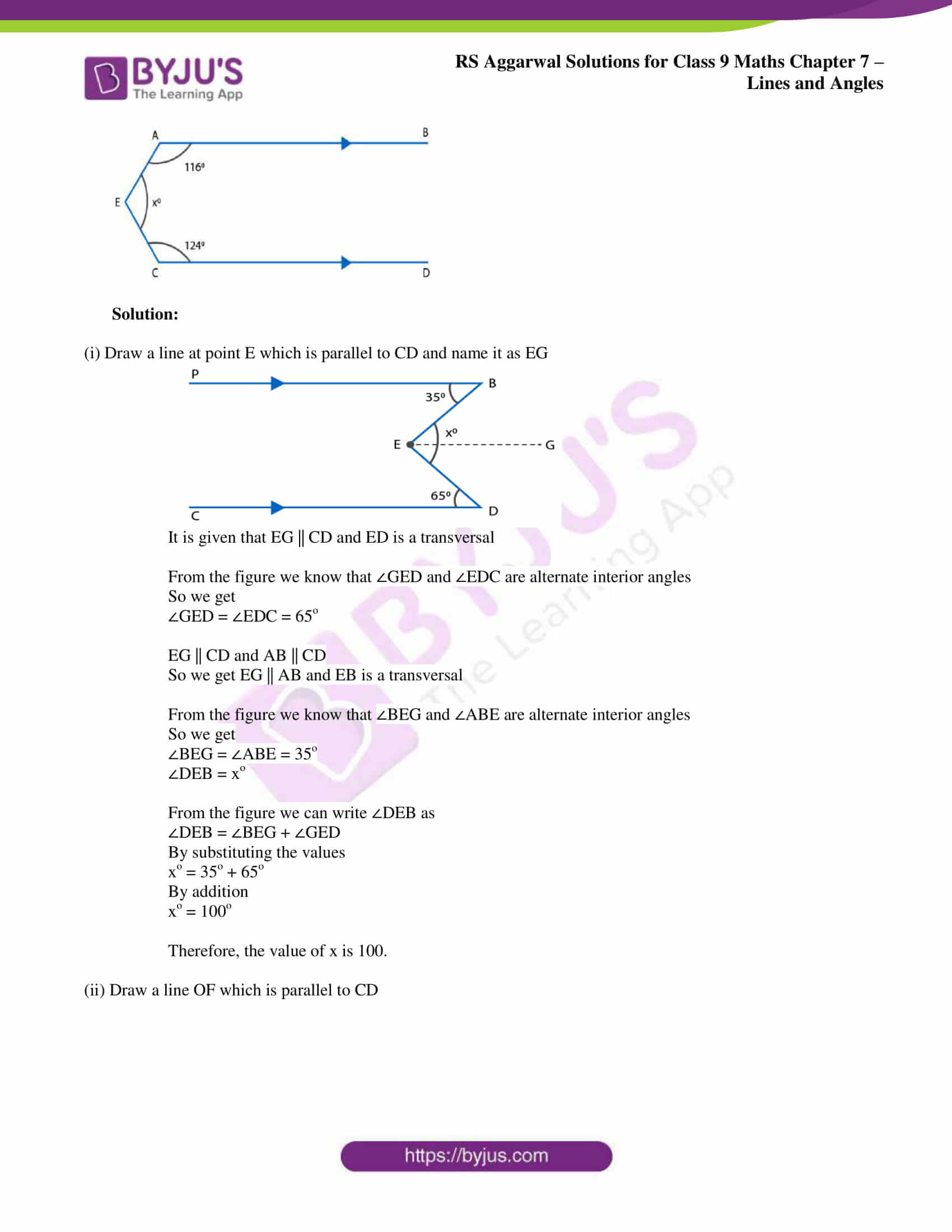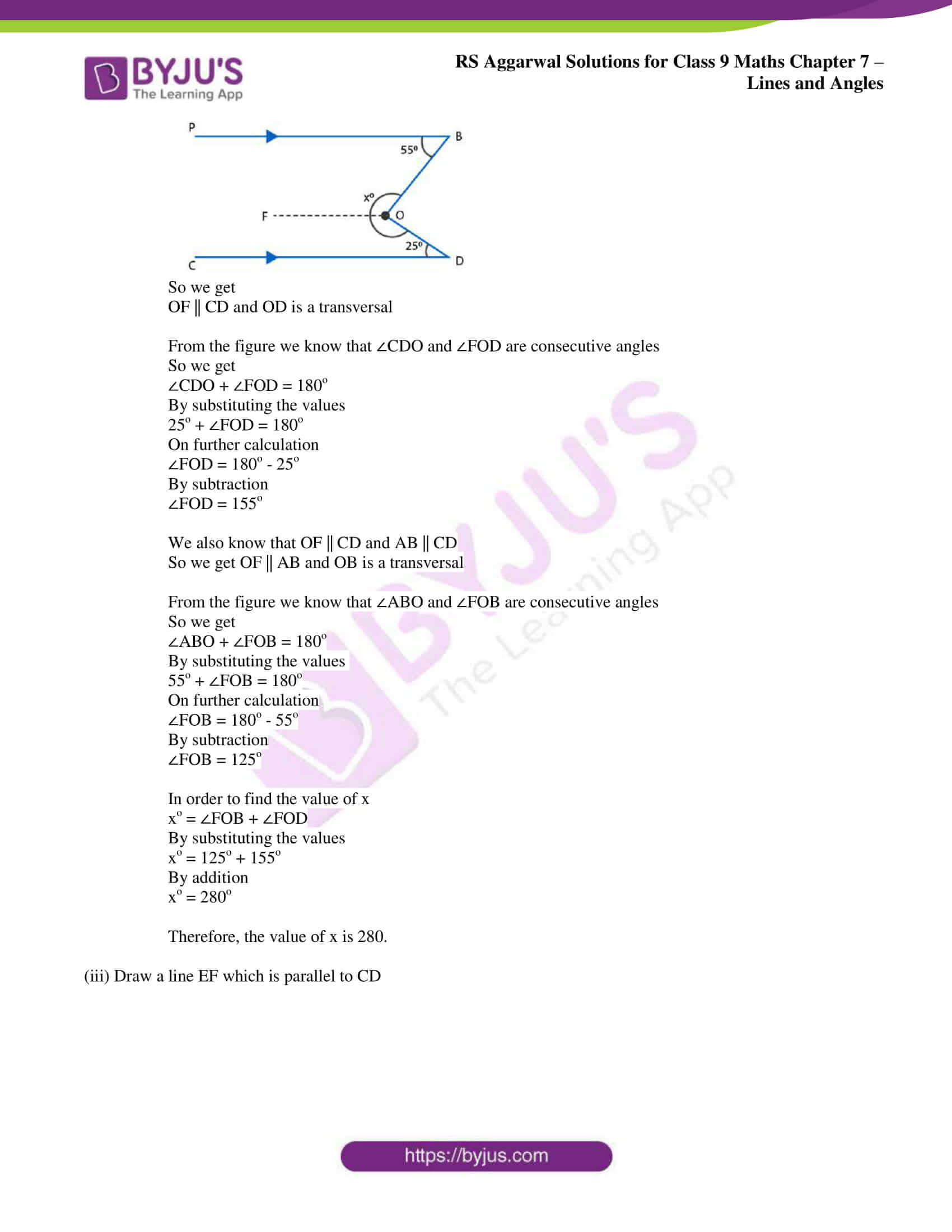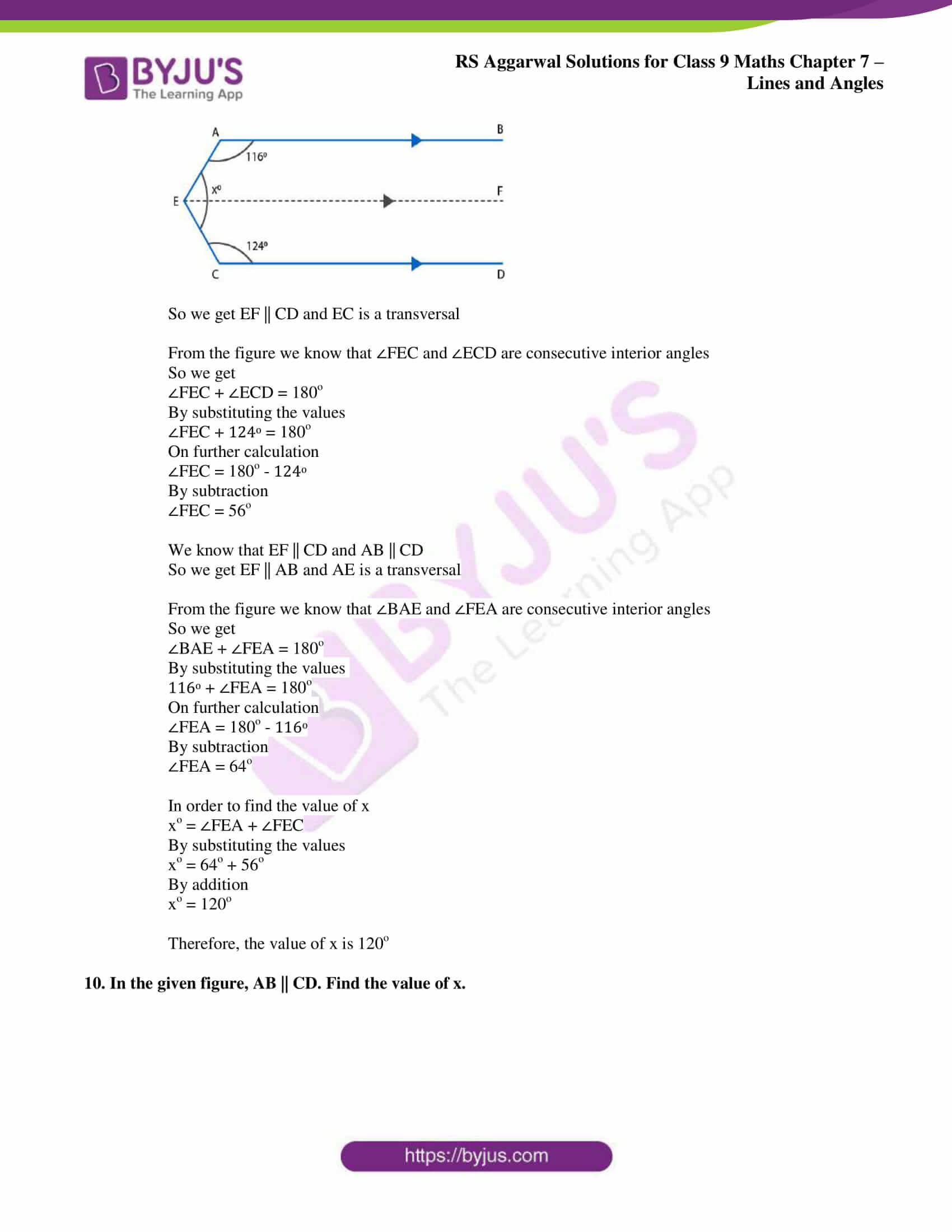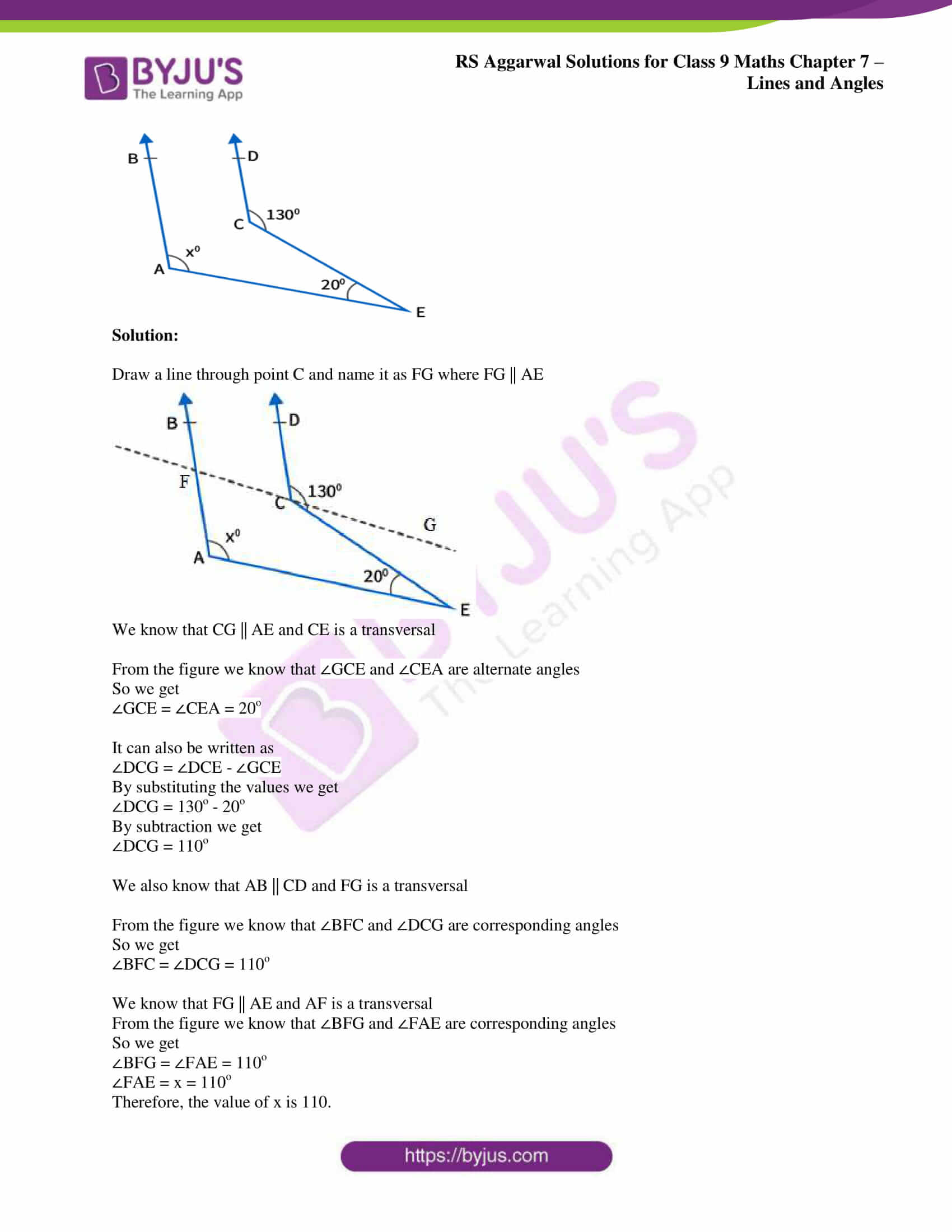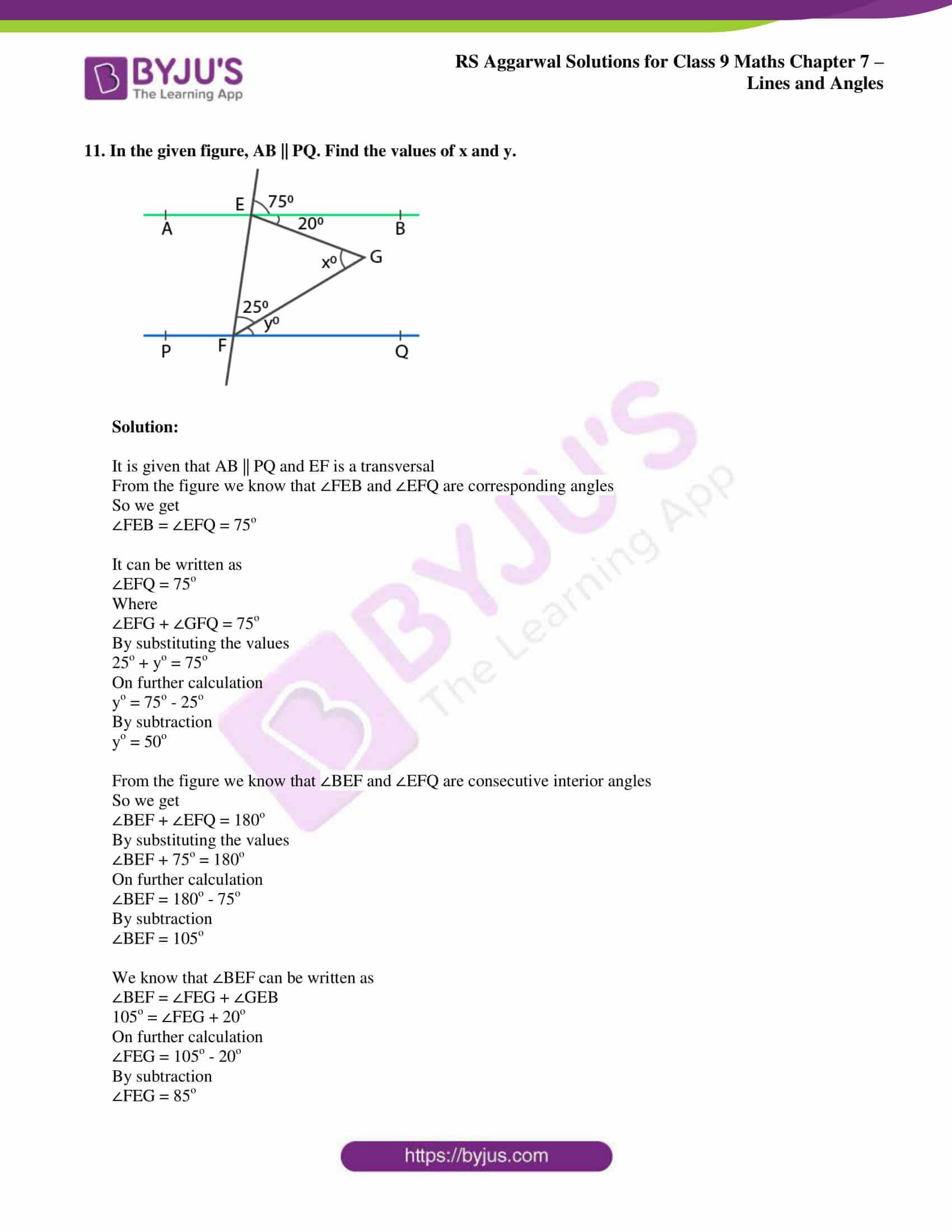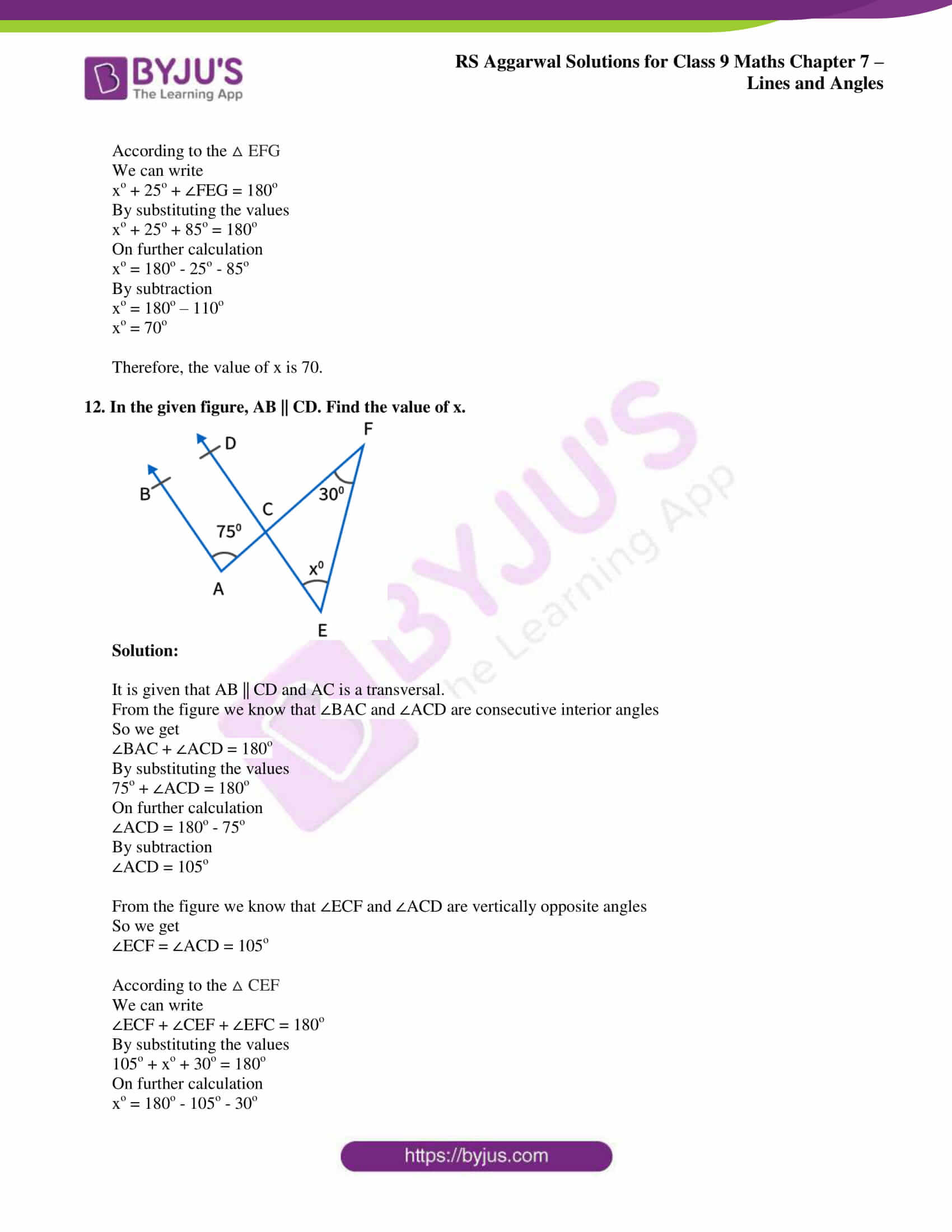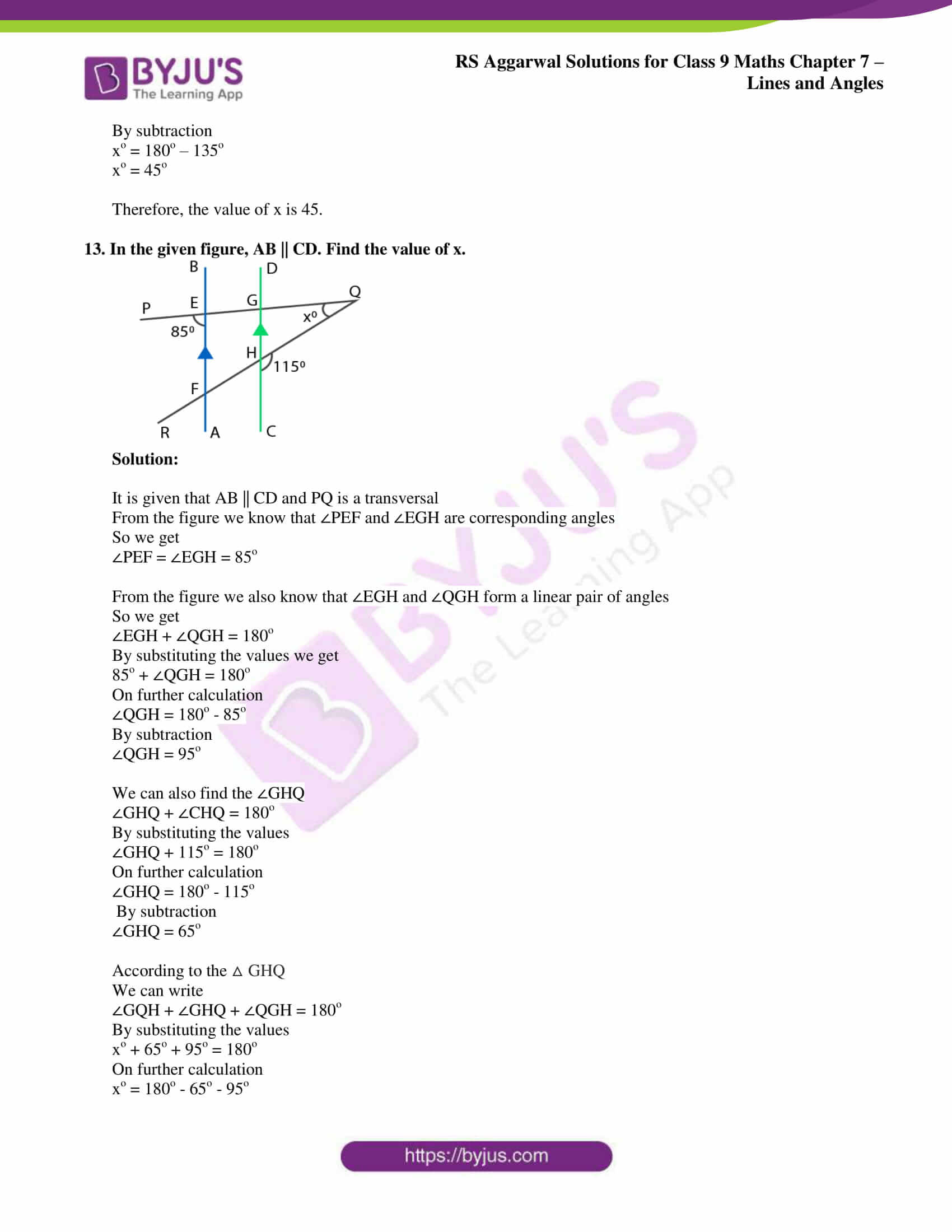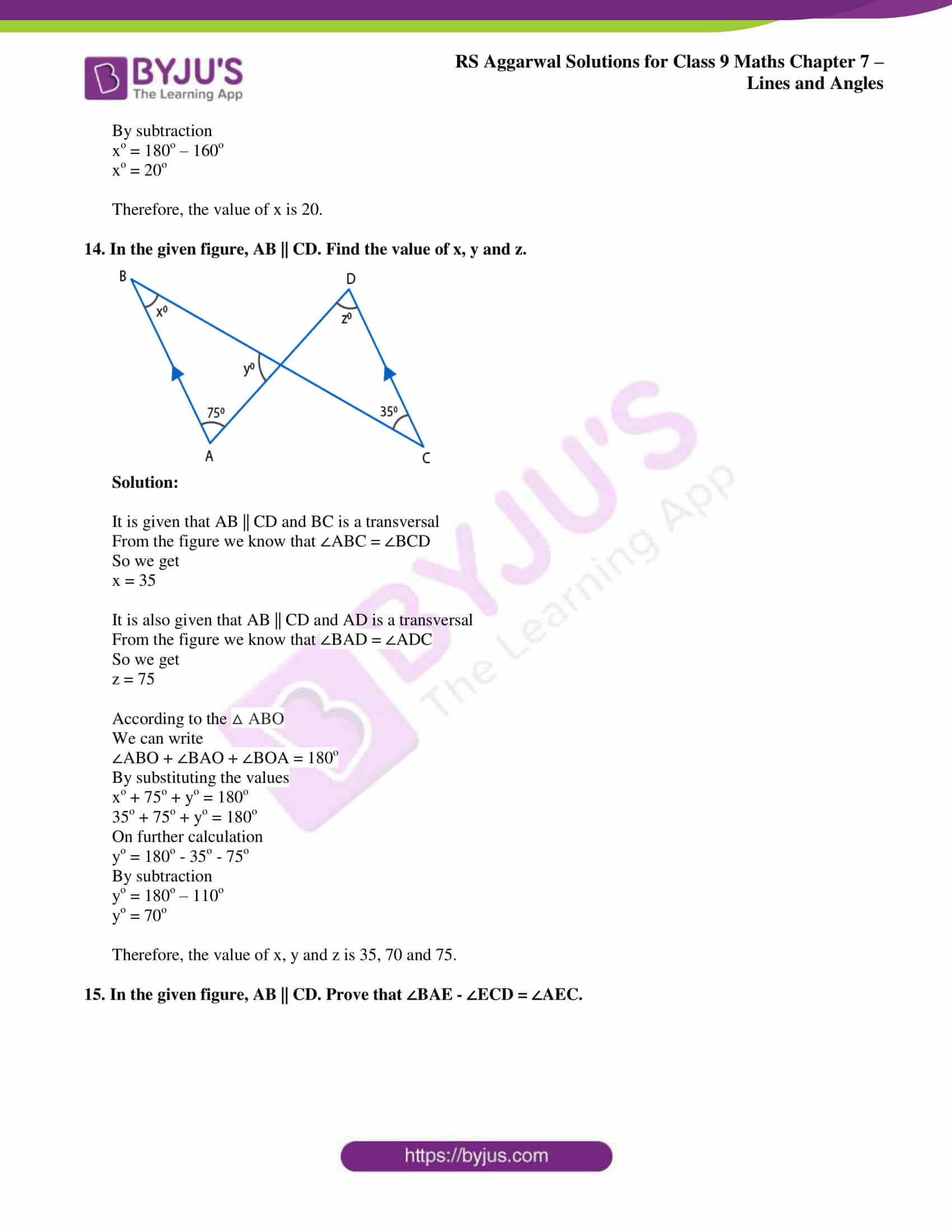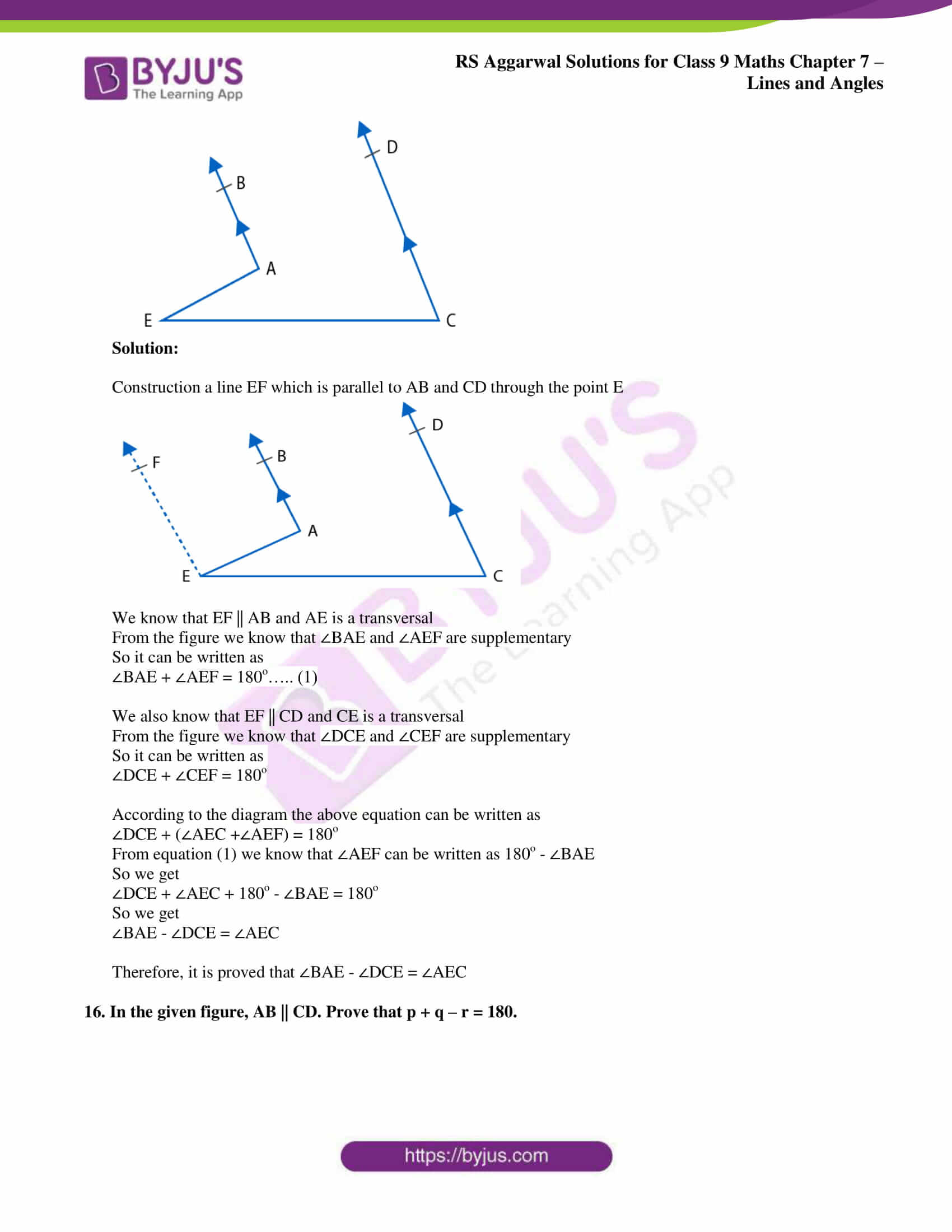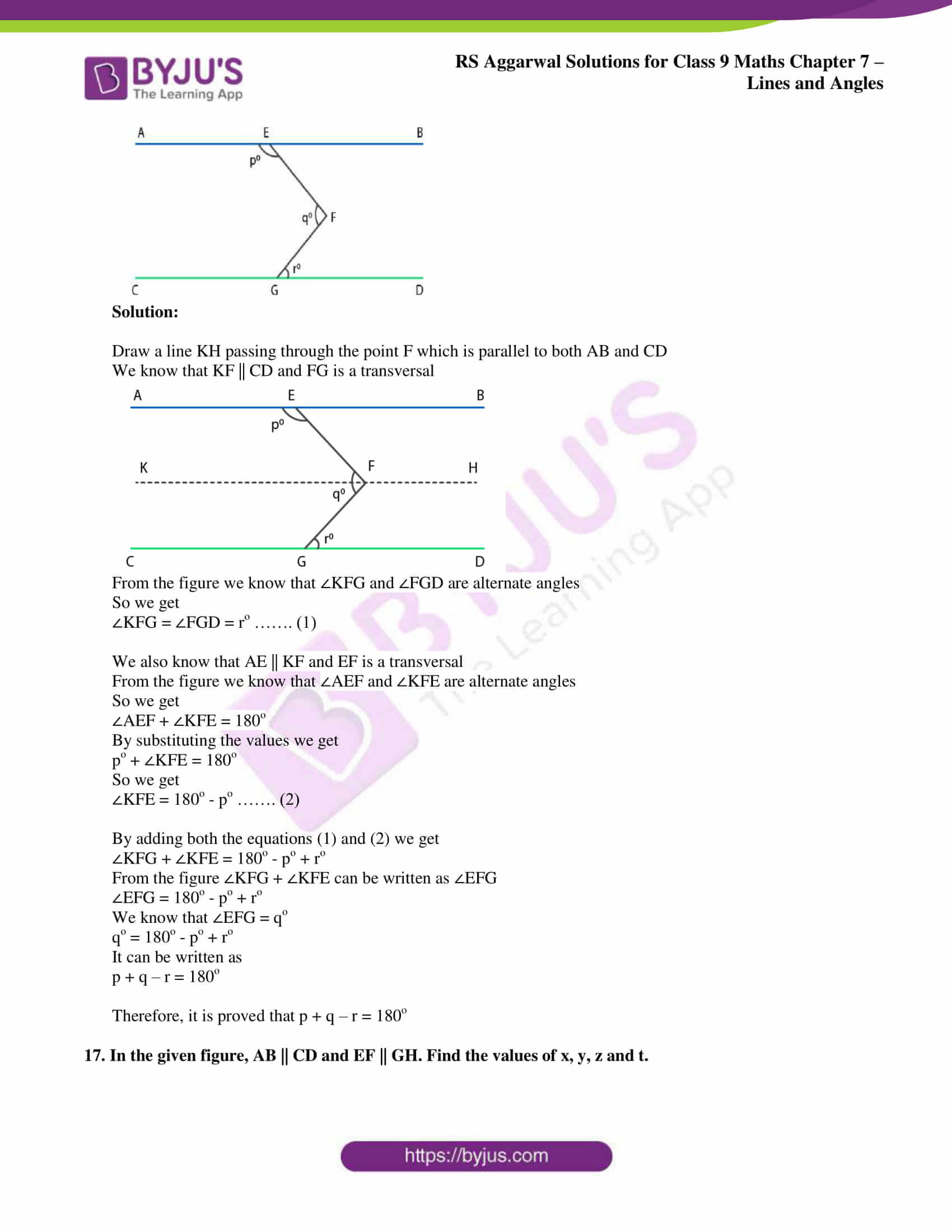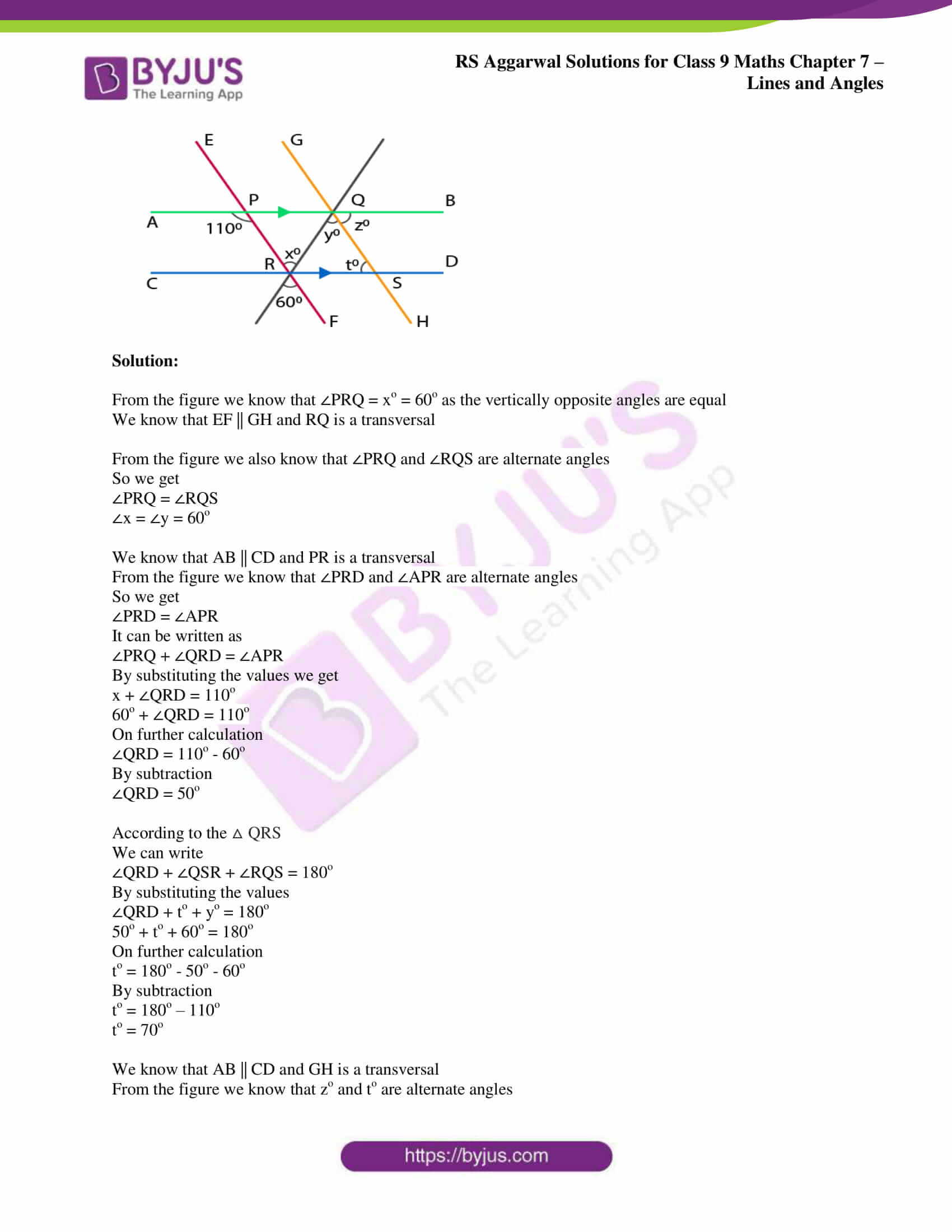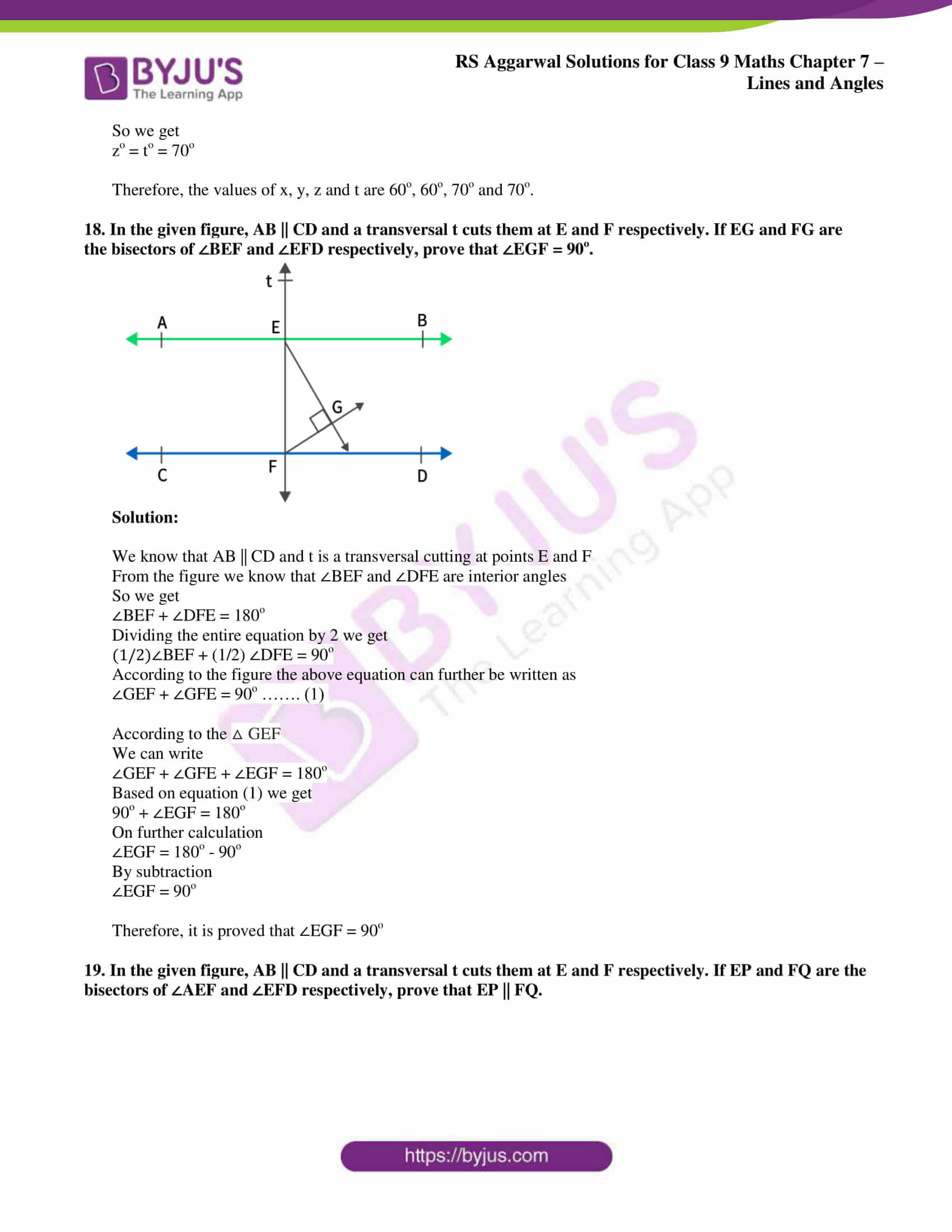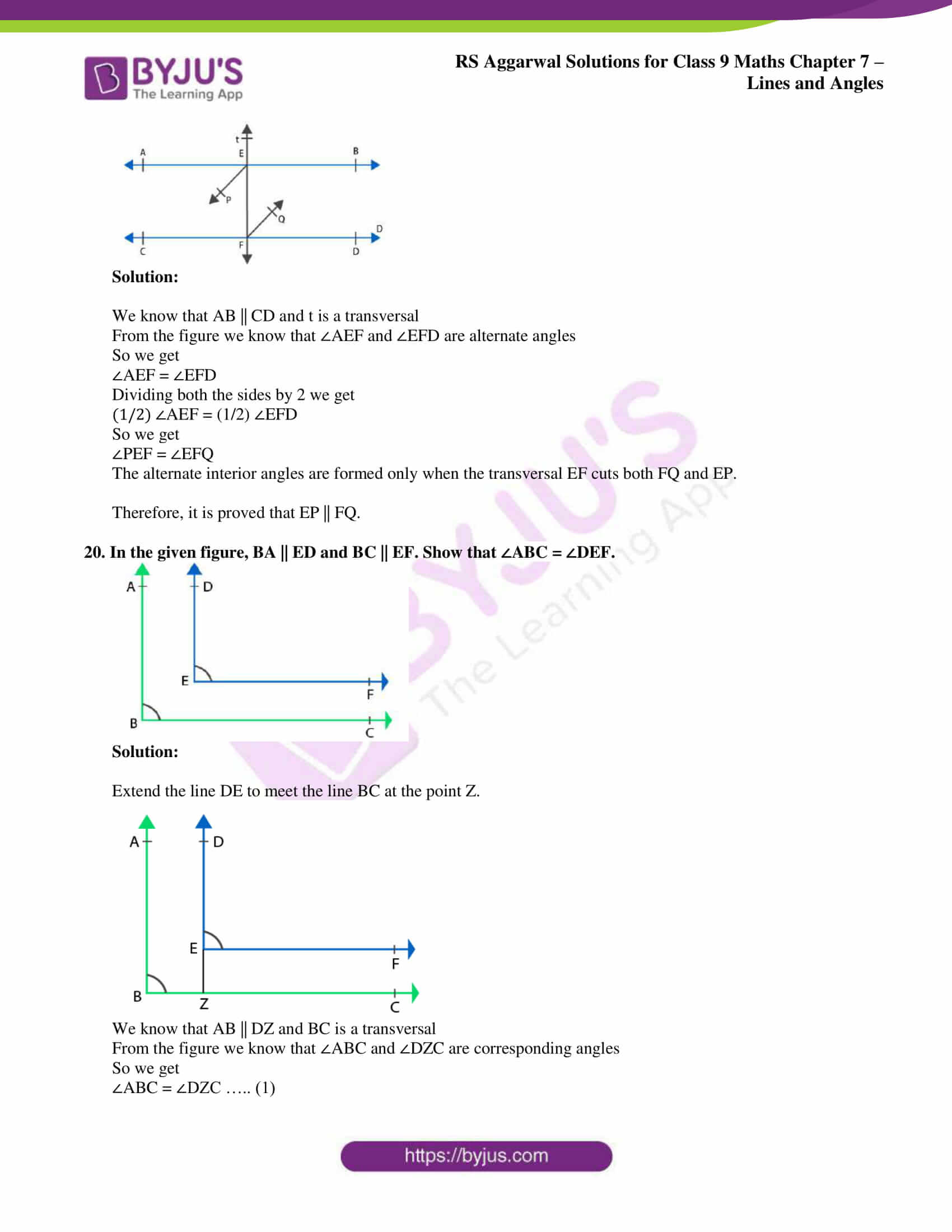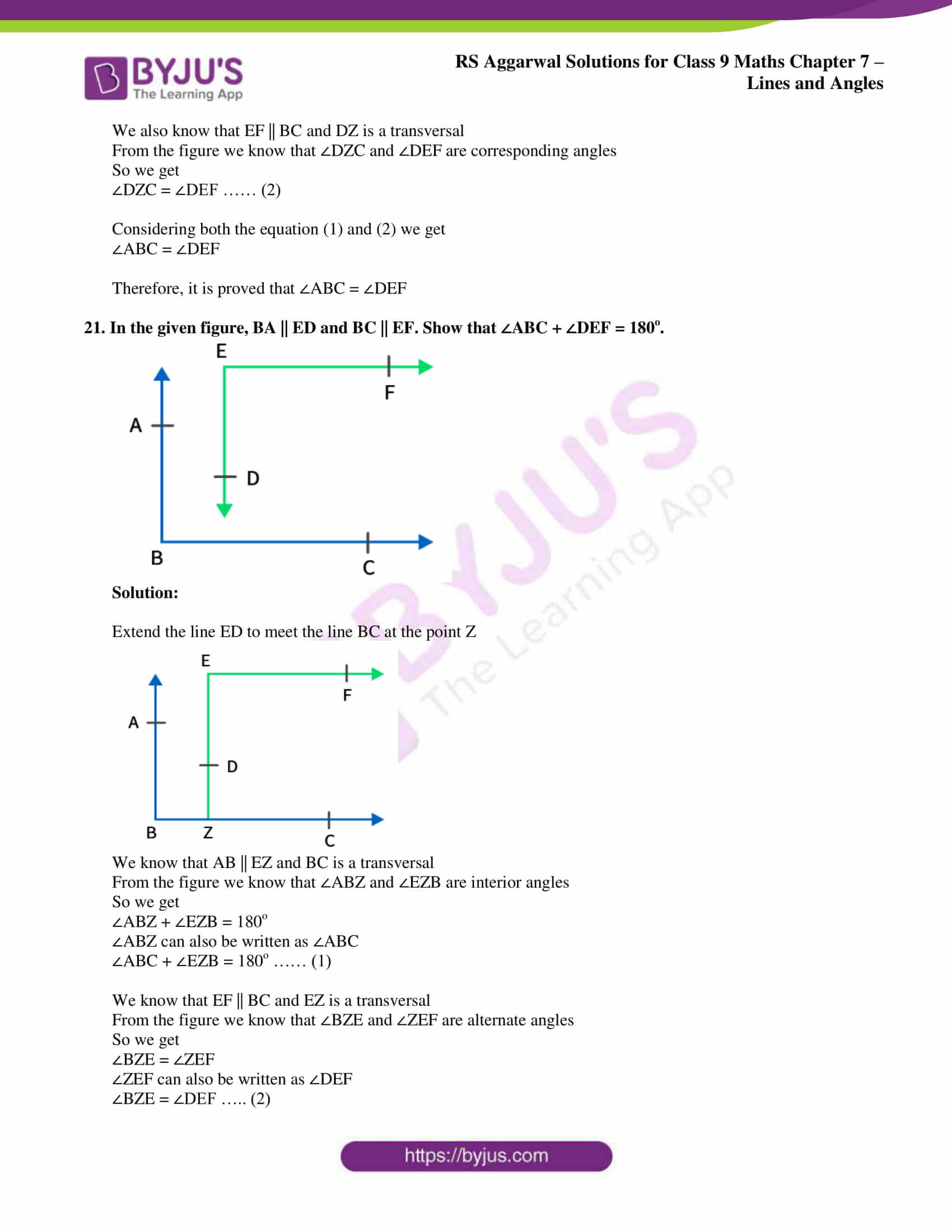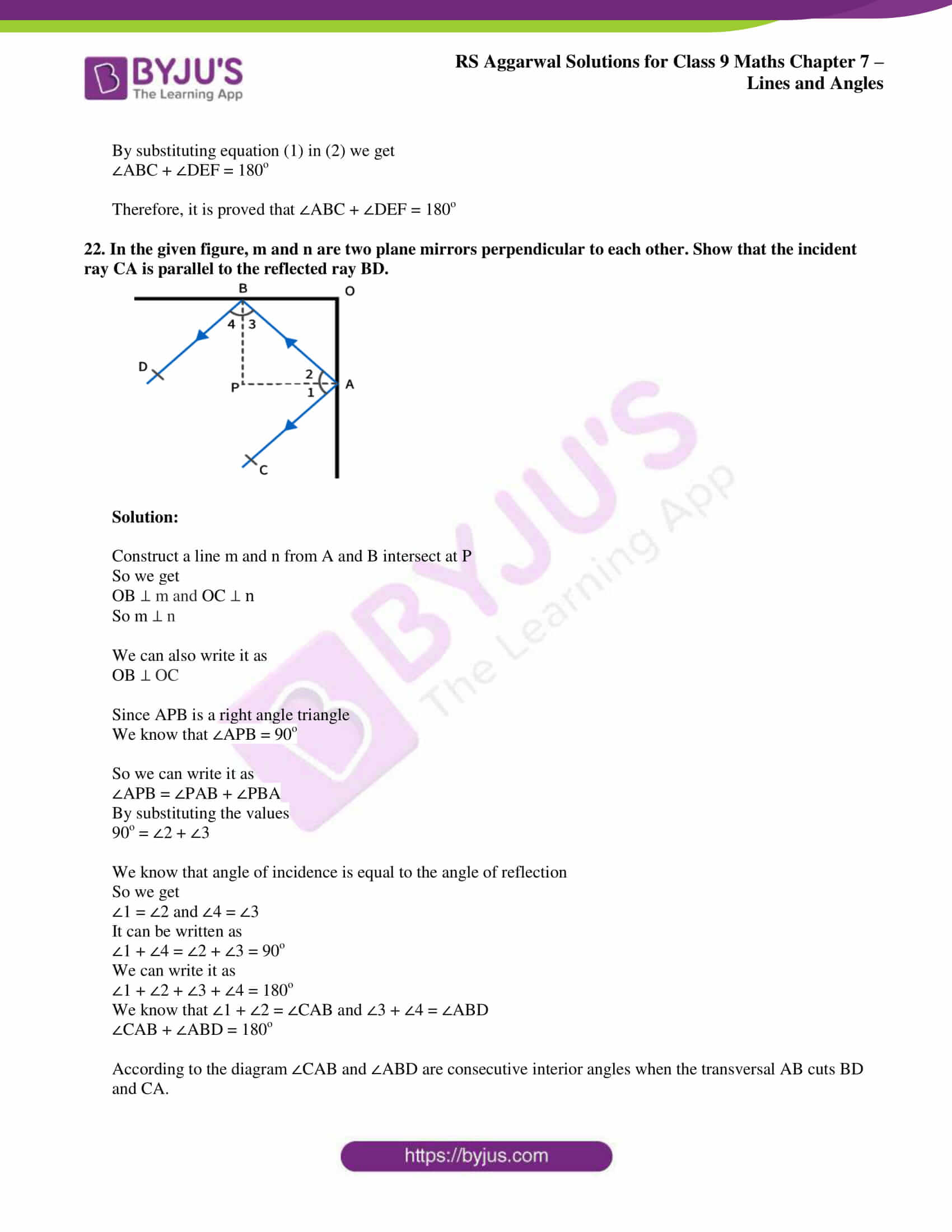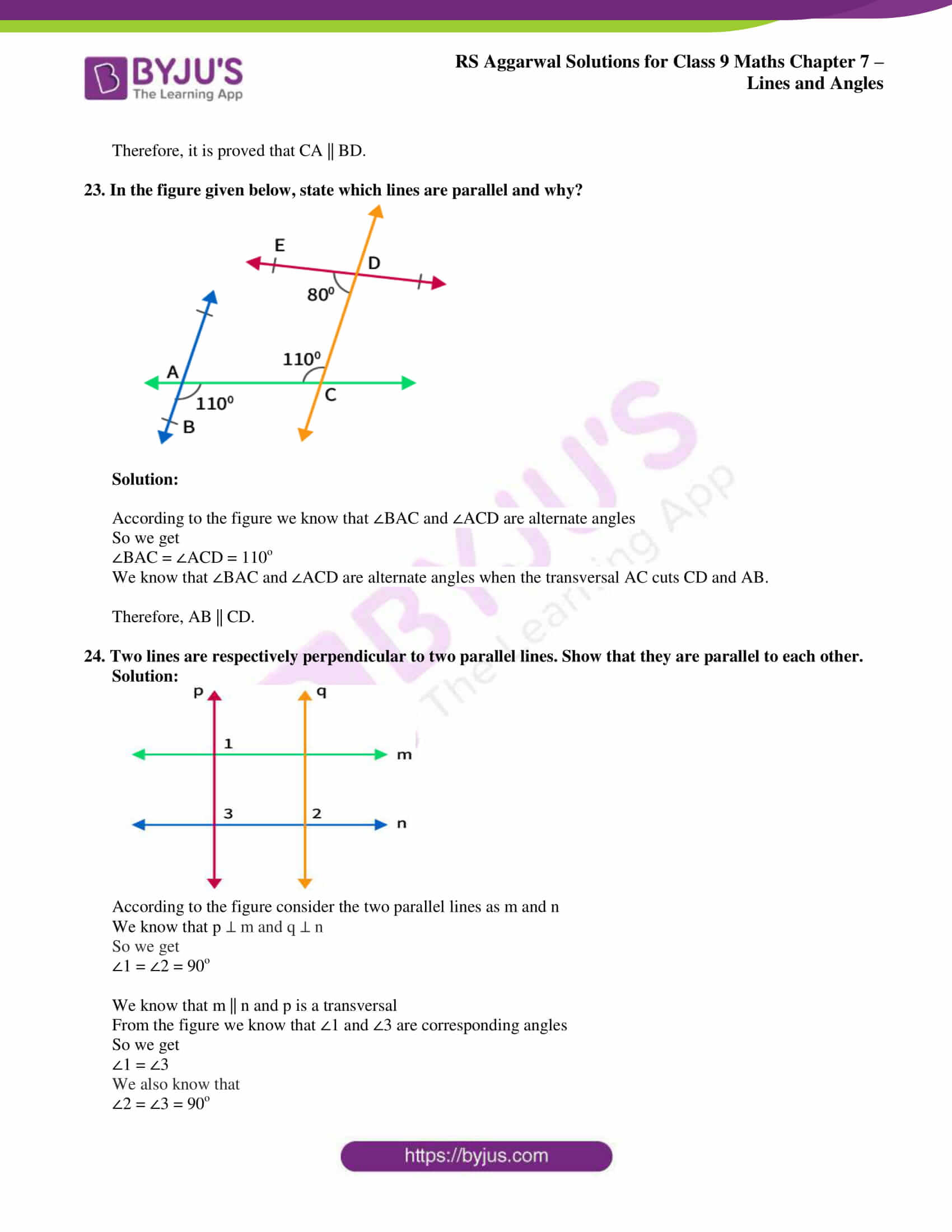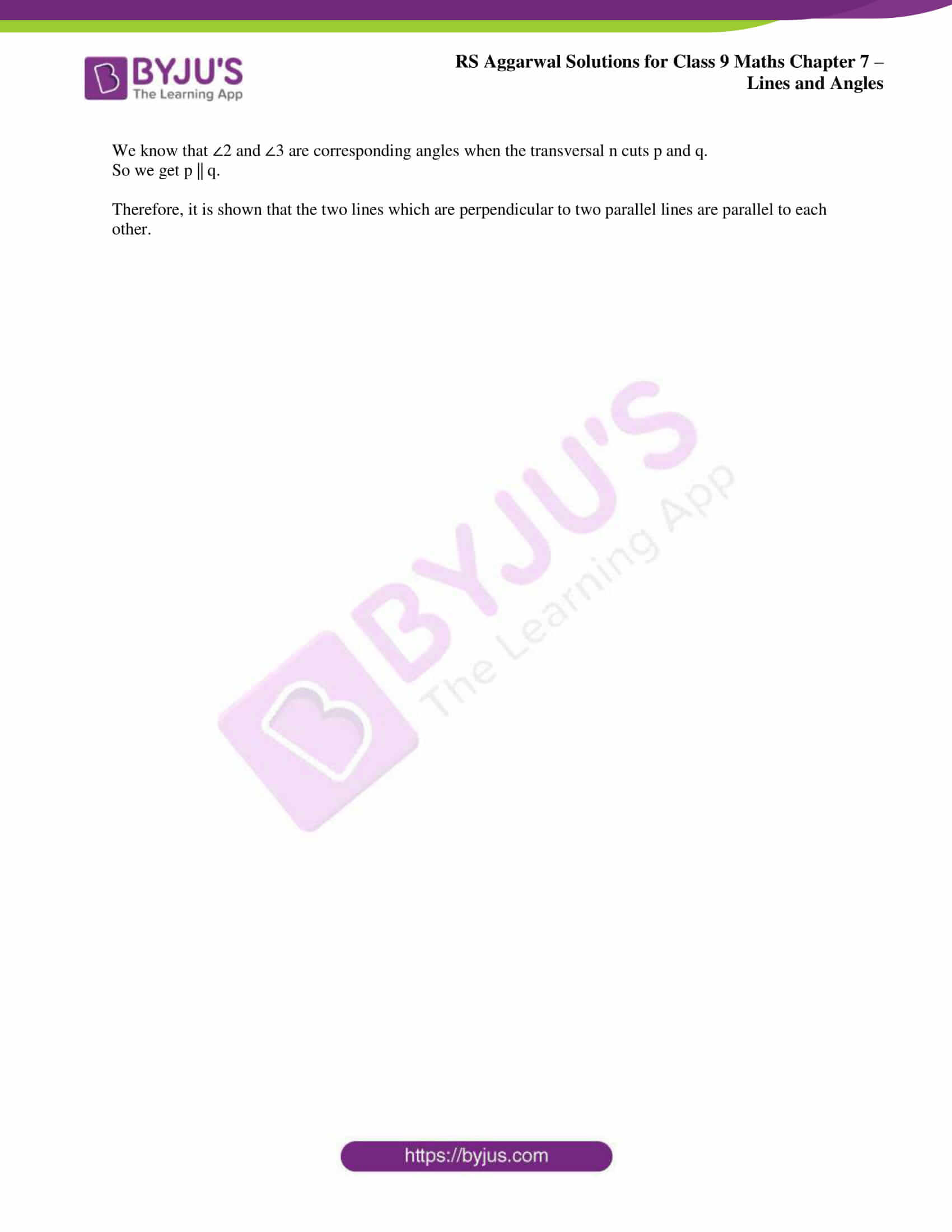## Access RS Aggarwal Solutions for Class 9 Maths Chapter 7: Lines and Angles

Exercise 7(A) PAGE: 198

1. Define the following terms:

(i) Angle

(ii) Interior of an angle

(iii) Obtuse angle

(iv) Reflex angle

(v) Complementary angles

(vi) Supplementary angles

Solution:

(i) Angle – When two rays originate from the same end point, then an angle is formed.

(ii) Interior of an angle – The interior of ∠BAC is the set of all points in its plane which lie on the same side of AB as C and also on the same side of AC as B.

(iii)Obtuse angle – An angle whose measure is more than 90o but less than 180o is called an obtuse angle.

(iv) Reflex angle – An angle whose measure is more than 180o but less than 360o is called a reflex angle.

(v) Complementary angles – Two angles are said to be complementary, if the sum of their measures is 90o.

(vi) Supplementary angles – Two angles are said to be supplementary if the sum of their measures is 180o.

2. Find the complement of each of the following angles:

(i) 55o

(ii) 16o

(iii) 90o

(iv) 2/3 of a right angle

Solution:

(i) We know that the complement of 55o can be written as

55o = 90o – 55o = 35o

(ii) We know that the complement of 16o can be written as

16o = 90o – 16o = 74o

(iii) We know that the complement of 90o can be written as

90o = 90o – 90o = 0o

(iv) We know that 2/3 of a right angle can be written as 2/3 × 90o = 60o

60o = 90o – 60o = 30o

3. Find the supplement of each of the following angles:

(i) 42o

(ii) 90o

(iii) 124o

(iv) 3/5 of a right angle

Solution:

(i) We know that the supplement of 42o can be written as

42o = 180o – 42o = 138o

(ii) We know that the supplement of 90o can be written as

90o = 180o – 90o = 90o

(iii) We know that the supplement of 124o can be written as

124o = 180o – 124o = 56o

(iv) We know that 3/5 of a right angle can be written as 3/5 × 90o = 54o

54o = 180o – 54o = 126o

4. Find the measure of an angle which is

(i) Equal to its complement

(ii) Equal to its supplement

Solution:

(i) Consider the required angle as xo

We know that the complement can be written as 90o – xo

To find that the measure of an angle is equal to its complement

We get

xo = 90o – xo

We can also write it as

x + x = 90

So we get

2x = 90

By division we get

x = 90/2

xo = 45o

Therefore, the measure of an angle which is equal to its complement is 45o

(ii) Consider the required angle as xo

We know that the supplement can be written as 180o – xo

To find that the measure of an angle is equal to its supplement

We get

xo = 180o – xo

We can also write it as

x + x = 180

So we get

2x = 180

By division we get

x = 180/2

xo = 90o

Therefore, the measure of an angle which is equal to its supplement is 90o

5. Find the measure of an angle which is 36o more than its complement.

Solution:

Consider the required angle as xo

We know that the complement can be written as 90o – xo

xo = (90o – xo) + 36o

We can also write it as

x + x = 90 + 36

So we get

2x = 126

By division we get

x = 126/2

xo = 63o

Therefore, the measure of an angle which is 36o more than its complement is 63o.

6. Find the measure of an angle which is 30o less than its supplement.

Solution:

Consider the required angle as xo

We know that the supplement can be written as 180o – xo

xo = (180o – xo) – 30o

We can also write it as

x + x = 180 – 30

So we get

2x = 150

By division we get

x = 150/2

xo = 75o

Therefore, the measure of an angle which is 30o less than its supplement is 75o.

7. Find the angle which is four times its complement.

Solution:

Consider the required angle as xo

We know that the complement can be written as 90o – xo

xo = 4(90o – xo)

We can also write it as

x = 360 – 4x

So we get

5x = 360

By division we get

x = 360/5

xo = 72o

Therefore, the angle which is four times its complement is 72o.

8. Find the angle which is five times its supplement.

Solution:

Consider the required angle as xo

We know that the supplement can be written as 180o – xo

xo = 5(180o – xo)

We can also write it as

x = 900 – 5x

So we get

6x = 900

By division we get

x = 900/6

xo = 150o

Therefore, the angle which is five times its supplement is 150o.

9. Find the angle whose supplement is four times its complement.

Solution:

Consider the required angle as xo

We know that the complement can be written as 90o – xo and the supplement can be written as 180o – xo

180o – xo = 4(90o – xo)

We can also write it as

180o – xo = 360o – 4xo

So we get

4xo – xo = 360o – 180o

3xo = 180o

By division we get

xo = 180/3

xo = 60o

Therefore, the angle whose supplement is four times its complement is 60o.

10. Find the angle whose complement is one third of its supplement.

Solution:

Consider the required angle as xo

We know that the complement can be written as 90o – xo and the supplement can be written as 180o – xo

90o – xo = 1/3(180o – xo)

We can also write it as

90o – xo = 60o – (1/3) xo

So we get

xo – (1/3)xo = 90o – 60o

(2/3) xo = 30o

By division we get

xo = ((30×3)/2)

xo = 45o

Therefore, the angle whose complement is one third of its supplement is 45o.

11. Two complementary angles are in the ratio 4:5. Find the angles.

Solution:

Consider the required angle as xo and 90o – xo

According to the question it can be written as

xo/ 90o – xo = 4/5

By cross multiplication we get

5x = 4 (90 – x)

5x = 360 – 4x

On further calculation we get

5x + 4x = 360

9x = 360

By division

x = 360/9

So we get

x = 40

Therefore, the angles are 40o and 90o – xo = 90o – 40o = 50o

12. Find the value of x for which the angles (2x – 5) o and (x – 10) o are the complementary angles.

Solution:

It is given that (2x – 5) o and (x – 10) o are the complementary angles.

So we can write it as

(2x – 5) o + (x – 10) o = 90o

2x – 5o + x – 10o = 90o

On further calculation

3x – 15o = 90o

So we get

3x = 105o

By division

x = 105/3

x = 35o

Therefore, the value of x for which the angles (2x – 5) o and (x – 10) o are the complementary angles is 35o.

Exercise 7(B) PAGE: 206

1. In the adjoining figure, AOB is a straight line. Find the value of x.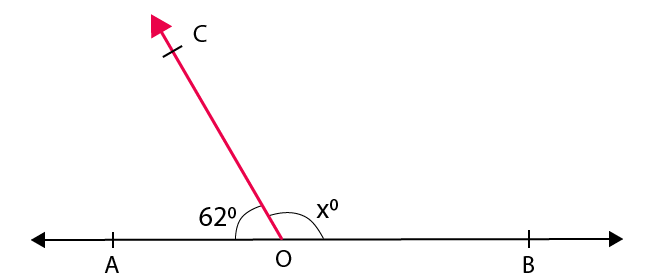Solution:

From the figure we know that ∠AOC and ∠BOC are a linear pair of angles

So we get

∠AOC + ∠BOC = 180o

We know that

62o + xo = 180o

On further calculation

xo = 180o – 62o

By subtraction

xo = 118o

Therefore, the value of x is 118.

2. In the adjoining figure, AOB is a straight line. Find the value of x. Hence, find ∠AOC and ∠BOD.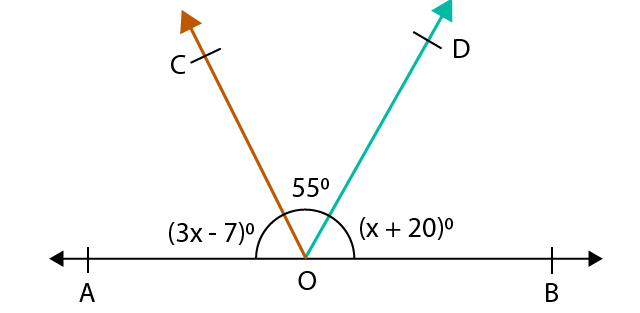Solution:

From the figure we know that ∠AOB is a straight line

So we get

∠AOB = 180o

It can also be written as

∠AOC + ∠COD + ∠BOD = 180o

By substituting the values

(3x – 7) o + 55o + (x + 20) o = 180o

3x – 7o + 55o + x + 20o = 180o

On further calculation

4x + 68o = 180o

4x = 112o

By division

x = 28o

By substituting the value of x we get

∠AOC = (3x – 7) o

= 3(28o) – 7o

On further calculation

= 84o – 7o

By subtraction

= 77o

∠BOD = (x + 20) o

= (28 + 20) o

= 48o

3. In the adjoining figure, AOB is a straight line. Find the value of x. Hence, find ∠AOC, ∠COD and ∠BOD.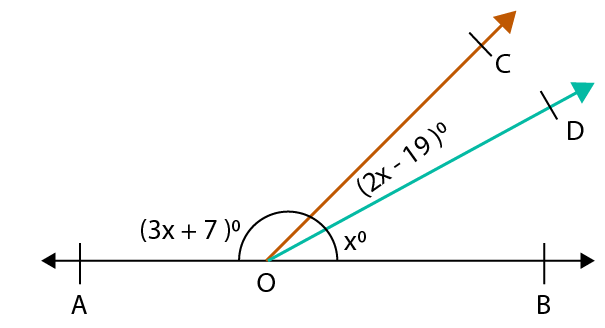Solution:

From the figure we know that ∠BOD and ∠AOD are a linear pair of angles

So we get

∠BOD + ∠AOD = 180o

It can also be written as

∠BOD + ∠COD + ∠COA = 180o

By substituting the values

xo + (2x – 19) o + (3x + 7) o = 180o

x + 2x – 19o + 3x + 7o = 180o

On further calculation

6x – 12o = 180o

6x = 180o + 12o

So we get

6x = 192o

By division

x = 32o

By substituting the value of x we get

∠AOC = (3x + 7) o

= 3(32o) + 7o

On further calculation

= 96o + 7o

= 103o

∠COD = (2x – 19) o

= (2(32o) – 19) o

So we get

= (64 – 19) o

By subtraction

= 45o

∠BOD = xo = 32o

4. In the adjoining figure, x: y: z = 5: 4: 6. If XOY is a straight line, find the values of x, y and z.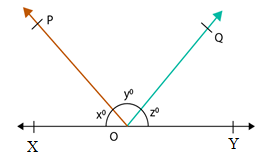Solution:

From the figure it is given that

x: y: z = 5: 4: 6

We can also write it as

x + y + z = 5 + 4 + 6 = 15

It is given that XOY is a straight line

So we know that

x + y + z = 180o

As we know the sum of ratios is 15 then we can write that the measure of x as 5

The sum of all the angles in a straight line is 180o

So we get the measure of x as

x = (5/15) × 180

On further calculation

x = 60

As we know the sum of ratios is 15 then we can write that the measure of y as 4

The sum of all the angles in a straight line is 180o

So we get the measure of y as

y = (4/15) × 180

On further calculation

y = 48

In order to find the value of z

We know that

x + y + z = 180o

Substituting the values of x and y we get

60o + 48o + z = 180o

On further calculation

z = 180o – 60o – 48o

By subtraction we get

z = 72o

Therefore, the values of x, y and z are 60o, 48o and 72o.

5. In the adjoining figure, what value of x will make AOB, a straight line?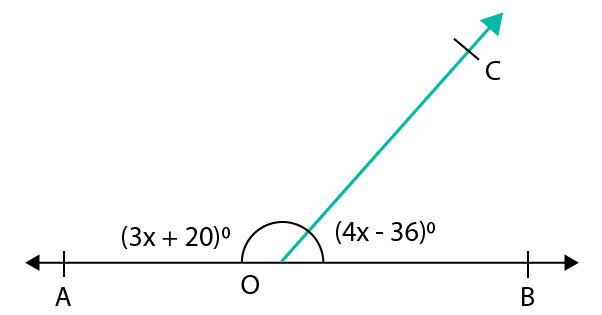Solution:

We know that AOB will be a straight line only if the adjacent angles form a linear pair.

∠BOC + ∠AOC = 180o

By substituting the values we get

(4x – 36) o + (3x + 20) o = 180o

4x – 36o + 3x + 20o = 180o

On further calculation we get

7x = 180o – 20o + 36o

7x = 196o

By division we get

x = 196/7

x = 28

Therefore, the value of x is 28.

6. Two lines AB and CD intersect at O. If ∠AOC = 50o, find ∠AOD, ∠BOD and ∠BOC.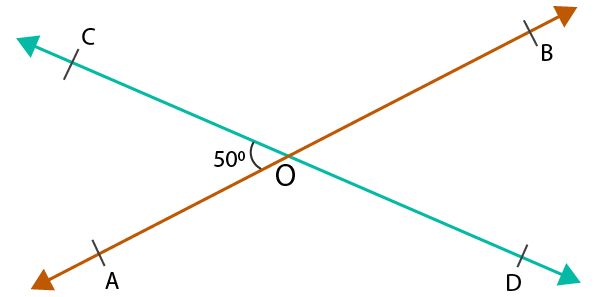Solution:

From the figure we know that ∠AOC and ∠AOD form a linear pair.

It can also be written as

∠AOC + ∠AOD = 180o

By substituting the values

50o + ∠AOD = 180o

∠AOD = 180o – 50o

By subtraction

∠AOD = 130o

According to the figure we know that ∠AOD and ∠BOC are vertically opposite angles

So we get

∠AOD = ∠BOC = 130o

According to the figure we know that ∠AOC and ∠BOD are vertically opposite angles

So we get

∠AOC = ∠BOD = 50o

7. In the adjoining figure, three coplanar lines AB, CD and EF intersect at a point O, forming angles as shown. Find the values of x, y, z and t.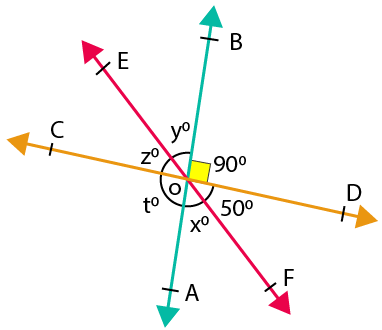Solution:

From the figure we know that ∠COE and ∠DOF are vertically opposite angles

∠COE = ∠DOF = ∠z = 50o

From the figure we know that ∠BOD and ∠COA are vertically opposite angles

∠BOD = ∠COA = ∠t = 90o

We also know that ∠COA and ∠AOD form a linear pair

∠COA + ∠AOD = 180o

It can also be written as

∠COA + ∠AOF + ∠FOD = 180o

By substituting values in the above equation we get

90o + xo + 50o = 180o

On further calculation we get

xo + 140o = 180o

xo = 180o – 140o

By subtraction

xo = 40o

From the figure we know that ∠EOB and ∠AOF are vertically opposite angles

∠EOB = ∠AOF = x = y = 40

Therefore, the values of x, y, z and t are 40, 40, 50 and 90.

8. In the adjoining figure, three coplanar lines AB, CD and EF intersect at a point O. Find the value of x. Hence, find ∠AOD, ∠COE and ∠AOE.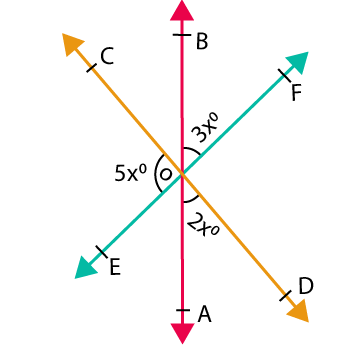Solution:

From the figure we know that ∠COE and ∠EOD form a linear pair

∠COE + ∠EOD = 180o

It can also be written as

∠COE + ∠EOA + ∠AOD = 180o

By substituting values in the above equation we get

5x + ∠EOA + 2x = 180o

From the figure we know that ∠EOA and ∠BOF are vertically opposite angles

∠EOA = ∠BOF

So we get

5x + ∠BOF + 2x = 180o

5x + 3x + 2x = 180o

On further calculation

10x = 180o

By division

x = 180/10 = 18

By substituting the value of x

∠AOD = 2xo

So we get

∠AOD = 2 (18) o = 36o

∠EOA = ∠BOF = 3xo

So we get

∠EOA = ∠BOF = 3 (18) o = 54o

∠COE = 5xo

So we get

∠COE = 5 (18) o = 90o

9. Two adjacent angles on a straight line are in the ratio 5:4. Find the measure of each one of these angles.

Solution:

Consider the two adjacent angles as 5x and 4x.

We know that the two adjacent angles form a linear pair

So it can be written as

5x + 4x = 180o

On further calculation

9x = 180o

By division

x = 180/9

x = 20o

Substituting the value of x in two adjacent angles

5x = 5 (20) o = 100o

4x = 4 (20) o = 80o

Therefore, the measure of each one of these angles is 100o and 80o

10. If two straight lines intersect each other in such a way that one of the angles formed measures 90o, show that each of the remaining angles measures 90o.

Solution:

Consider two lines AB and CD intersecting at a point O with ∠AOC = 90o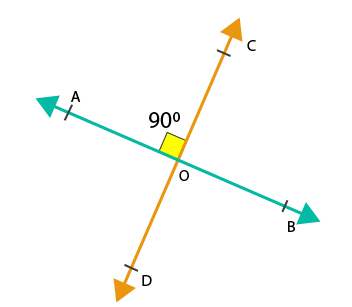From the figure we know that ∠AOC and ∠BOD are vertically opposite angles

∠AOC = ∠BOD = 90o

From the figure we also know that ∠AOC and ∠AOD form a linear pair

So it can be written as

∠AOC + ∠AOD = 180o

Substituting the values

90o + ∠AOD = 180o

On further calculation

∠AOD = 180o – 90o

By subtraction

∠AOD = 90o

From the figure we also know that ∠BOC and ∠AOD are vertically opposite angles

∠BOC = ∠AOD = 90o

Therefore, it is proved that each of the remaining angles is 90o

11. Two lines AB and CD intersect at a point O such that ∠BOC + ∠AOD = 280o, as shown in the figure. Find all the four angles.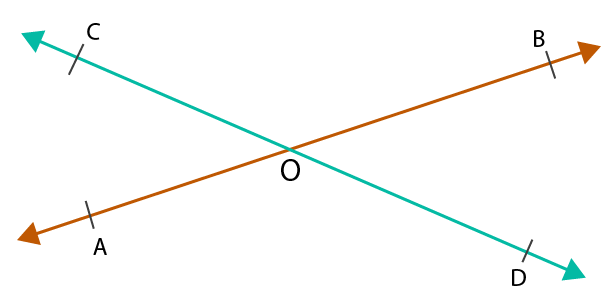Solution:

From the figure we know that ∠AOD and ∠BOC are vertically opposite angles

∠AOD = ∠BOC

It is given that

∠BOC + ∠AOD = 280o

We know that ∠AOD = ∠BOC

So it can be written as

∠AOD + ∠AOD = 280o

On further calculation

2 ∠AOD = 280o

By division

∠AOD = 280/2

∠AOD = ∠BOC = 140o

From the figure we know that ∠AOC and ∠AOD form a linear pair

So it can be written as

∠AOC + ∠AOD = 180o

Substituting the values

∠AOC + 140o = 180o

On further calculation

∠AOC = 180o – 140o

By subtraction

∠AOC = 40o

From the figure we know that ∠AOC and ∠BOD are vertically opposite angles

∠AOC = ∠BOD = 40o

Therefore, ∠AOC = 40o, ∠BOC = 140o, ∠AOD = 140o and ∠BOD = 40o

12. Two lines AB and CD intersect each other at a point O such that ∠AOC: ∠AOD = 5:7. Find all the angles.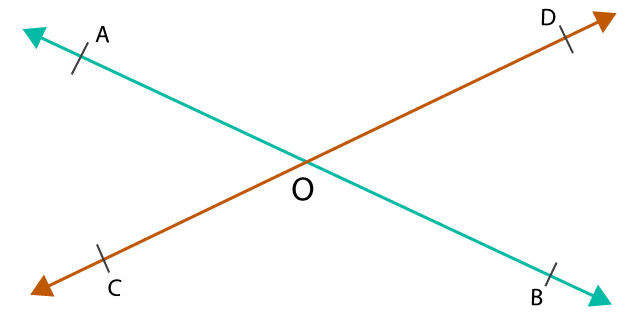Solution:

Consider ∠AOC as 5x and ∠AOD as 7x

From the figure we know that ∠AOC and ∠AOD for linear pair of angles

So it can be written as

∠AOC + ∠AOD = 180o

By substituting the values

5x + 7x = 180o

On further calculation

12x = 180o

By division

x = 180/12

x = 15o

By substituting the value of x

∠AOC = 5x

So we get

∠AOC = 5 (15o) = 75o

∠AOD = 7x

So we get

∠AOD = 7 (15o) = 105o

From the figure we know that ∠AOC and ∠BOD are vertical angles

So we get

∠AOC = ∠BOD = 75o

From the figure we know that ∠AOD and ∠BOC are vertical angles

So we get

∠AOD = ∠BOC = 105o

13. In the given figure, three lines AB, CD and EF intersect at a point O such that ∠AOE = 35o and ∠BOD = 40o. Find the measure of ∠AOC, ∠BOF, ∠COF and ∠DOE.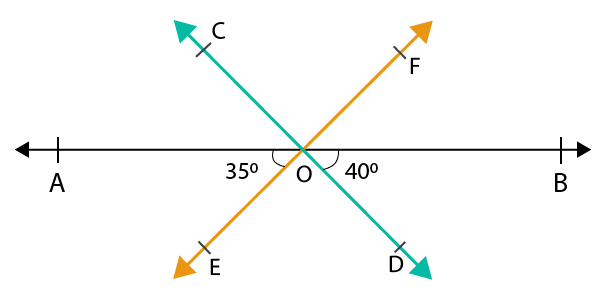Solution:

It is given that ∠BOD = 40o

From the figure we know that ∠BOD and ∠AOC are vertically opposite angles

∠AOC = ∠BOD = 40o

It is given that ∠AOE = 35o

From the figure we know that ∠BOF and ∠AOE are vertically opposite angles

∠AOE = ∠BOF = 35o

From the figure we know that AOB is a straight line

So it can be written as

∠AOB = 180o

We can write it as

∠AOE + ∠EOD + ∠BOD = 180o

By substituting the values

35o + ∠EOD + 40o = 180o

On further calculation

∠EOD = 180o – 35o – 40o

By subtraction

∠EOD = 105o

From the figure we know that ∠COF and ∠EOD are vertically opposite angles

∠COF = ∠EOD = 105o

14. In the given figure, the two lines AB and CD intersect at a point O such that ∠BOC = 125o. Find the values of x, y and z.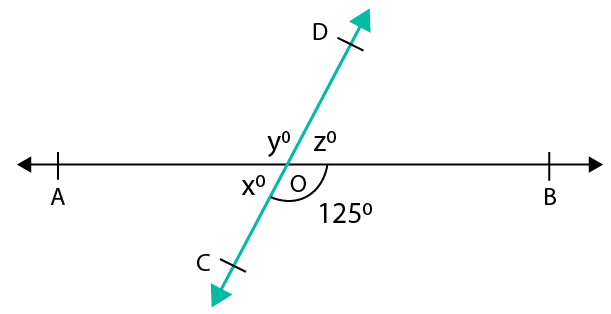Solution:

From the figure we know that ∠AOC and ∠BOC form a linear pair of angles

So it can be written as

∠AOC + ∠BOC = 180o

By substituting the values we get

x + 125o = 180o

On further calculation

x = 180o – 125o

By subtraction

x = 55o

From the figure we know that ∠AOD and ∠BOC are vertically opposite angles

So we get

y = 125o

From the figure we know that ∠BOD and ∠AOC are vertically opposite angles

So we get

z = 55o

Therefore, the values of x, y and z are 55o, 125o and 55o

15. If two straight lines intersect each other than prove that the ray opposite to the bisector of one of the angles so formed bisects the vertically opposite angle.

Solution:

Given: Let us consider AB and CD as the two lines intersecting at a point O where OE is the ray bisecting ∠BOD and OF is the ray bisecting ∠AOC.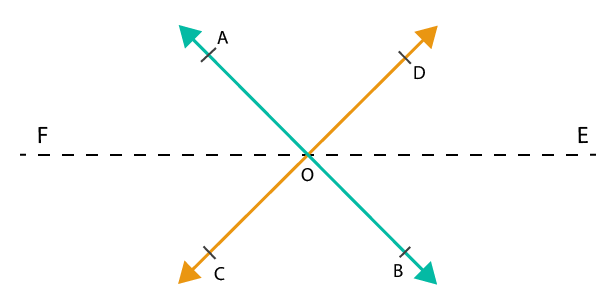To Prove: ∠AOF = ∠COF

Proof: EF is a straight line passing through the point O where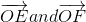are two opposite rays.

From the figure we know that ∠AOF and ∠BOE, ∠COF and ∠DOE are vertically opposite angles

So it can be written as

∠AOF = ∠BOE and ∠COF = ∠DOE

It is given that ∠BOE = ∠DOE

So we can write it as ∠AOF = ∠COF

Therefore, it is proved that ∠AOF = ∠COF.

16. Prove that the bisectors of two adjacent supplementary angles include a right angle.

Solution:

Given: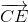is the bisector of ∠ACD and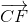is the bisector of ∠BCD

To Prove: ∠ECF = 90o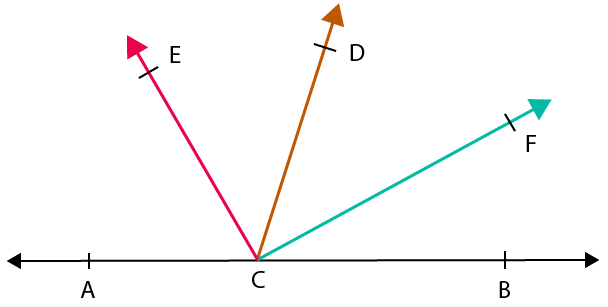Proof:

From the figure we know that

∠ACD and ∠BCD form a linear pair of angles

So we can write it as

∠ACD + ∠BCD = 180o

We can also write it as

∠ACE + ∠ECD + ∠DCF + ∠FCB = 180o

From the figure we also know that

∠ACE = ∠ECD and ∠DCF = ∠FCB

So it can be written as

∠ECD + ∠ECD + ∠DCF + ∠DCF = 180o

On further calculation we get

2 ∠ECD + 2 ∠DCF = 180o

Taking out 2 as common we get

2 (∠ECD + ∠DCF) = 180o

By division we get

(∠ECD + ∠DCF) = 180/2

∠ECD + ∠DCF = 90o

Therefore, it is proved that ∠ECF = 90o

Exercise 7(C) PAGE: 223

1. In the given figure, l || m and a transversal t cuts them. If ∠1 = 120o, find the measure of each of the remaining marked angles.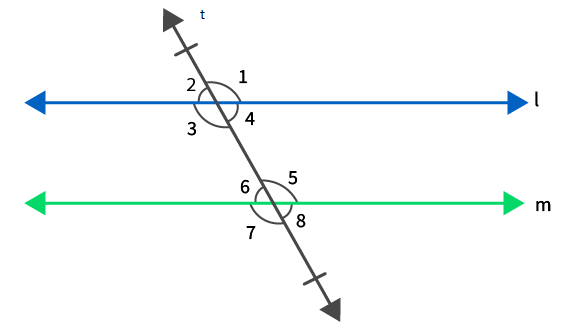Solution:

It is given that ∠1 = 120o

From the figure we know that ∠1 and ∠2 form a linear pair of angles

So it can be written as

∠1 + ∠2 = 180o

By substituting the values

120o + ∠2 = 180o

On further calculation

∠2 = 180o – 120o

By subtraction

∠2 = 60o

From the figure we know that ∠1 and ∠3 are vertically opposite angles

So we get

∠1 = ∠3 = 120o

From the figure we know that ∠2 and ∠4 are vertically opposite angles

So we get

∠2 = ∠4 = 60o

It is given that, l || m and t is a transversal

So the corresponding angles according to the figure is written as

∠1 = ∠5 = 120o

∠2 = ∠6 = 60o

∠3 = ∠7 = 120o

∠4 = ∠8 = 60o

2. In the figure, l || m and a transversal t cuts them. If ∠7 = 80o, find the measure of each of the remaining marked angles.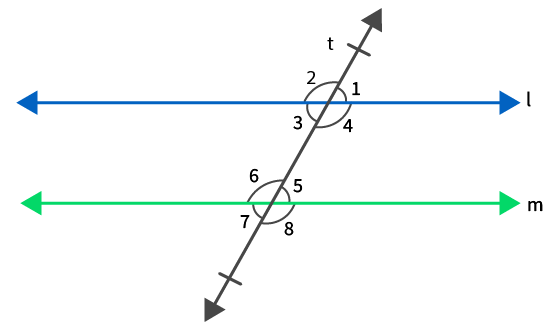Solution:

It is given that ∠7 = 80o

From the figure we know that ∠7 and ∠8 form a linear pair of angles

So it can be written as

∠7 + ∠8 = 180o

By substituting the values

80o + ∠8 = 180o

On further calculation

∠8 = 180o – 80o

By subtraction

∠8 = 100o

From the figure we know that ∠7 and ∠5 are vertically opposite angles

So we get

∠7 = ∠5 = 80o

From the figure we know that ∠6 and ∠8 are vertically opposite angles

So we get

∠6 = ∠8 = 100o

It is given that, l || m and t is a transversal

So the corresponding angles according to the figure is written as

∠1 = ∠5 = 80o

∠2 = ∠6 = 100o

∠3 = ∠7 = 80o

∠4 = ∠8 = 100o

3. In the figure, l || m and a transversal t cuts them. If ∠1: ∠2 = 2: 3, find the measure of each of the marked angles.Solution:

It is given that ∠1: ∠2 = 2: 3

From the figure we know that ∠1 and ∠2 form a linear pair of angles

So it can be written as

∠1 + ∠2 = 180o

By substituting the values

2x + 3x = 180o

On further calculation

5x = 180o

By division

x = 180o/5

x = 36o

By substituting the value of x we get

∠1 = 2x = 2 (36o) = 72o

∠2 = 3x = 3 (36o) = 108o

From the figure we know that ∠1 and ∠3 are vertically opposite angles

So we get

∠1 = ∠3 = 72o

From the figure we know that ∠2 and ∠4 are vertically opposite angles

So we get

∠2 = ∠4 = 108o

It is given that, l || m and t is a transversal

So the corresponding angles according to the figure is written as

∠1 = ∠5 = 72o

∠2 = ∠6 = 108o

∠3 = ∠7 = 72o

∠4 = ∠8 = 108o

4. For what value of x will the lines l and m be parallel to each other?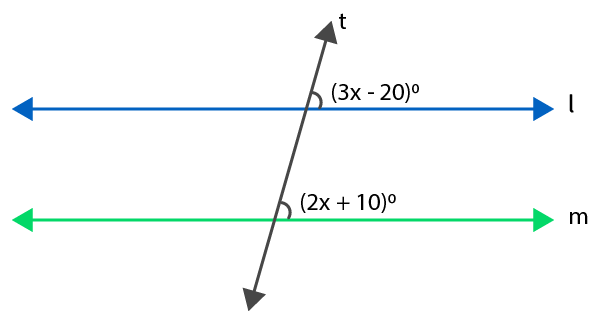Solution:

If the lines l and m are parallel it can be written as

3x – 20 = 2x + 10

We know that the two lines are parallel if the corresponding angles are equal

3x – 2x = 10 + 20

On further calculation

x = 30

Therefore, the value of x is 30.

5. For what value of x will the lines l and m be parallel to each other?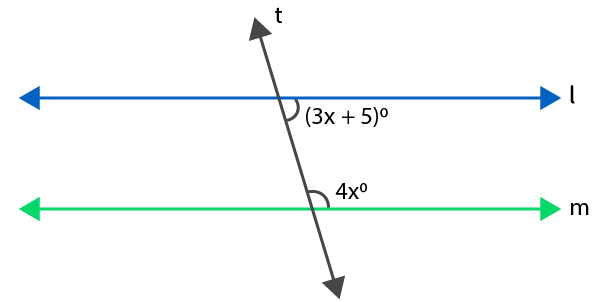Solution:

We know that both the angles are consecutive interior angles

So it can be written as

3x + 5 + 4x = 180

On further calculation we get

7x = 180 – 5

By subtraction

7x = 175

By division we get

x = 175/ 7

x = 25

Therefore, the value of x is 25.

6. In the figure, AB || CD and BC || ED. Find the value of x.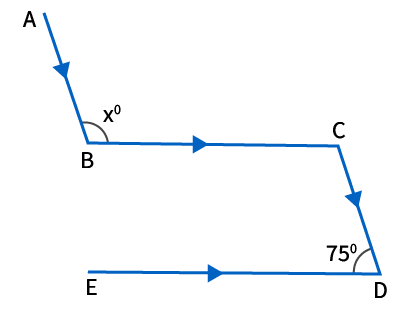Solution:

From the given figure we know that

AB and CD are parallel line and BC is a transversal

We know that ∠BCD and ∠ABC are alternate angles

So we can write it as

∠BCD = ∠ABC = xo

We also know that BC || ED and CD is a transversal

From the figure we know that ∠BCD and ∠EDC form a linear pair of angles

So it can be written as

∠BCD + ∠EDC = 180o

By substituting the values we get

∠BCD + 75o = 180o

On further calculation we get

∠BCD = 180o – 75o

By subtraction

∠BCD = 105o

From the figure we know that ∠BCD and ∠ABC are alternate angles

So we get

∠BCD = ∠ABC = x = 105o

∠ABC = x = 105o

Therefore, the value of x is 105o.

7. In the figure, AB || CD || EF. Find the value of x.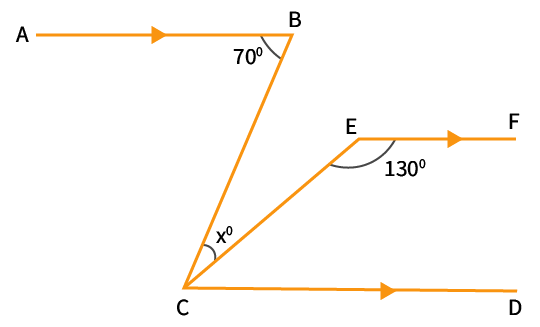Solution:

It is given that AB || CD and BC is a transversal

From the figure we know that ∠BCD and ∠ABC are alternate interior angles

So we get

∠ABC = ∠BCD

In order to find the value of x we can write it as

xo + ∠ECD = 70o ……. (1)

It is given that CD || EF and CE is a transversal

From the figure we know that ∠ECD and ∠CEF are consecutive interior angles

So we get

∠ECD + ∠CEF = 180o

By substituting the values

∠ECD + 130o = 180o

On further calculation we get

∠ECD = 180o – 130o

By subtraction

∠ECD = 50o

Now by substituting ∠ECD in equation (1) we get

xo + ∠ECD = 70o

xo + 50o = 70o

On further calculation we get

xo = 70o – 50o

By subtraction

xo = 20o

Therefore, the value of x is 20o.

8. In the given figure, AB || CD. Find the values of x, y and z.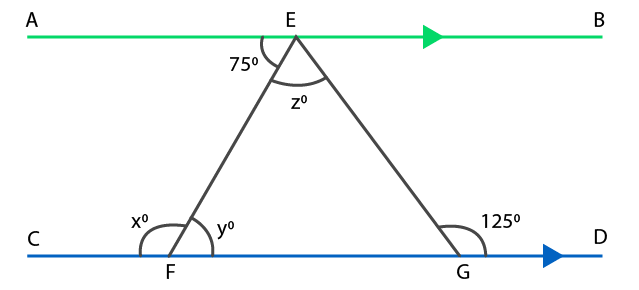Solution:

It is given that AB || CD and EF is a transversal

From the figure we know that ∠AEF and ∠EFG are alternate angles

So we get

∠AEF = ∠EFG = 75o

∠EFG = y = 75o

From the figure we know that ∠EFC and ∠EFG form a linear pair of angles

So we get

∠EFC + ∠EFG = 180o

It can also be written as

x + y = 180o

By substituting the value of y we get

x + 75o = 180o

On further calculation we get

x = 180o – 75o

By subtraction

x = 105o

From the figure based on the exterior angle property it can be written as

∠EGD = ∠EFG + ∠FEG

By substituting the values in the above equation we get

125o = y + z

125o = 75o + z

On further calculation we get

z = 125o – 75o

By subtraction

z = 50o

Therefore, the values of x, y and z are 105o, 75o and 50o.

9. In each of the figures given below, AB || CD. Find the value of x in each case.

(i)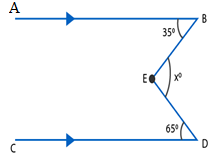(ii)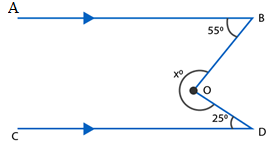(iii)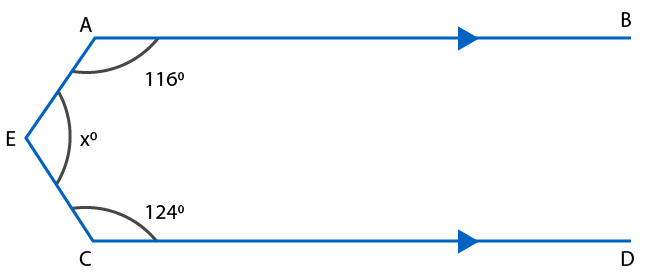Solution:

(i) Draw a line at point E which is parallel to CD and name it as EG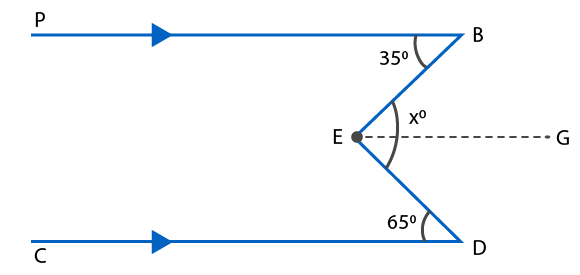It is given that EG || CD and ED is a transversal

From the figure we know that ∠GED and ∠EDC are alternate interior angles

So we get

∠GED = ∠EDC = 65o

EG || CD and AB || CD

So we get EG || AB and EB is a transversal

From the figure we know that ∠BEG and ∠ABE are alternate interior angles

So we get

∠BEG = ∠ABE = 35o

∠DEB = xo

From the figure we can write ∠DEB as

∠DEB = ∠BEG + ∠GED

By substituting the values

xo = 35o + 65o

xo = 100o

Therefore, the value of x is 100.

(ii) Draw a line OF which is parallel to CD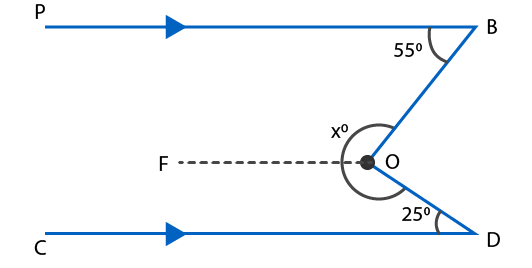So we get

OF || CD and OD is a transversal

From the figure we know that ∠CDO and ∠FOD are consecutive angles

So we get

∠CDO + ∠FOD = 180o

By substituting the values

25o + ∠FOD = 180o

On further calculation

∠FOD = 180o – 25o

By subtraction

∠FOD = 155o

We also know that OF || CD and AB || CD

So we get OF || AB and OB is a transversal

From the figure we know that ∠ABO and ∠FOB are consecutive angles

So we get

∠ABO + ∠FOB = 180o

By substituting the values

55o + ∠FOB = 180o

On further calculation

∠FOB = 180o – 55o

By subtraction

∠FOB = 125o

In order to find the value of x

xo = ∠FOB + ∠FOD

By substituting the values

xo = 125o + 155o

xo = 280o

Therefore, the value of x is 280.

(iii) Draw a line EF which is parallel to CD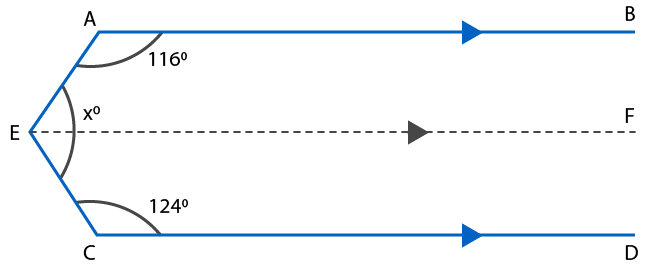So we get EF || CD and EC is a transversal

From the figure we know that ∠FEC and ∠ECD are consecutive interior angles

So we get

∠FEC + ∠ECD = 180o

By substituting the values

∠FEC + 124o = 180o

On further calculation

∠FEC = 180o – 124o

By subtraction

∠FEC = 56o

We know that EF || CD and AB || CD

So we get EF || AB and AE is a transversal

From the figure we know that ∠BAE and ∠FEA are consecutive interior angles

So we get

∠BAE + ∠FEA = 180o

By substituting the values

116o + ∠FEA = 180o

On further calculation

∠FEA = 180o – 116o

By subtraction

∠FEA = 64o

In order to find the value of x

xo = ∠FEA + ∠FEC

By substituting the values

xo = 64o + 56o

xo = 120o

Therefore, the value of x is 120o

10. In the given figure, AB || CD. Find the value of x.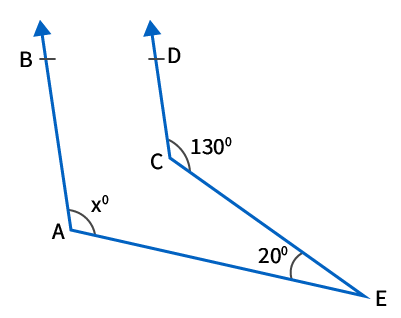Solution:

Draw a line through point C and name it as FG where FG || AE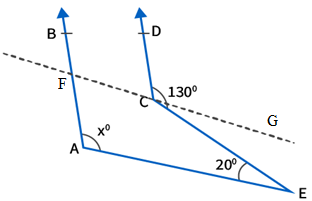We know that CG || AE and CE is a transversal

From the figure we know that ∠GCE and ∠CEA are alternate angles

So we get

∠GCE = ∠CEA = 20o

It can also be written as

∠DCG = ∠DCE – ∠GCE

By substituting the values we get

∠DCG = 130o – 20o

By subtraction we get

∠DCG = 110o

We also know that AB || CD and FG is a transversal

From the figure we know that ∠BFC and ∠DCG are corresponding angles

So we get

∠BFC = ∠DCG = 110o

We know that FG || AE and AF is a transversal

From the figure we know that ∠BFG and ∠FAE are corresponding angles

So we get

∠BFG = ∠FAE = 110o

∠FAE = x = 110o

Therefore, the value of x is 110.

11. In the given figure, AB || PQ. Find the values of x and y.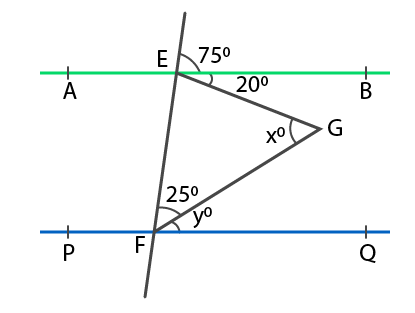Solution:

It is given that AB || PQ and EF is a transversal

From the figure we know that ∠FEB and ∠EFQ are corresponding angles

So we get

∠FEB = ∠EFQ = 75o

It can be written as

∠EFQ = 75o

Where

∠EFG + ∠GFQ = 75o

By substituting the values

25o + yo = 75o

On further calculation

yo = 75o – 25o

By subtraction

yo = 50o

From the figure we know that ∠BEF and ∠EFQ are consecutive interior angles

So we get

∠BEF + ∠EFQ = 180o

By substituting the values

∠BEF + 75o = 180o

On further calculation

∠BEF = 180o – 75o

By subtraction

∠BEF = 105o

We know that ∠BEF can be written as

∠BEF = ∠FEG + ∠GEB

105o = ∠FEG + 20o

On further calculation

∠FEG = 105o – 20o

By subtraction

∠FEG = 85o

According to the △ EFG

We can write

xo + 25o + ∠FEG = 180o

By substituting the values

xo + 25o + 85o = 180o

On further calculation

xo = 180o – 25o – 85o

By subtraction

xo = 180o – 110o

xo = 70o

Therefore, the value of x is 70.

12. In the given figure, AB || CD. Find the value of x.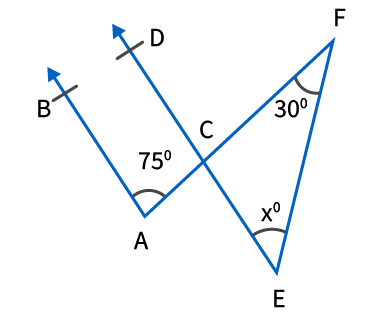Solution:

It is given that AB || CD and AC is a transversal.

From the figure we know that ∠BAC and ∠ACD are consecutive interior angles

So we get

∠BAC + ∠ACD = 180o

By substituting the values

75o + ∠ACD = 180o

On further calculation

∠ACD = 180o – 75o

By subtraction

∠ACD = 105o

From the figure we know that ∠ECF and ∠ACD are vertically opposite angles

So we get

∠ECF = ∠ACD = 105o

According to the △ CEF

We can write

∠ECF + ∠CEF + ∠EFC = 180o

By substituting the values

105o + xo + 30o = 180o

On further calculation

xo = 180o – 105o – 30o

By subtraction

xo = 180o – 135o

xo = 45o

Therefore, the value of x is 45.

13. In the given figure, AB || CD. Find the value of x.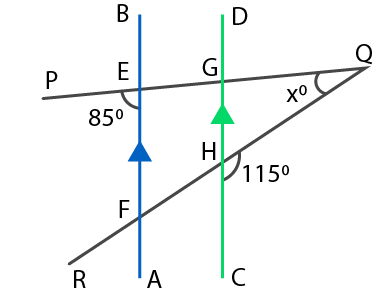Solution:

It is given that AB || CD and PQ is a transversal

From the figure we know that ∠PEF and ∠EGH are corresponding angles

So we get

∠PEF = ∠EGH = 85o

From the figure we also know that ∠EGH and ∠QGH form a linear pair of angles

So we get

∠EGH + ∠QGH = 180o

By substituting the values we get

85o + ∠QGH = 180o

On further calculation

∠QGH = 180o – 85o

By subtraction

∠QGH = 95o

We can also find the ∠GHQ

∠GHQ + ∠CHQ = 180o

By substituting the values

∠GHQ + 115o = 180o

On further calculation

∠GHQ = 180o – 115o

By subtraction

∠GHQ = 65o

According to the △ GHQ

We can write

∠GQH + ∠GHQ + ∠QGH = 180o

By substituting the values

xo + 65o + 95o = 180o

On further calculation

xo = 180o – 65o – 95o

By subtraction

xo = 180o – 160o

xo = 20o

Therefore, the value of x is 20.

14. In the given figure, AB || CD. Find the value of x, y and z.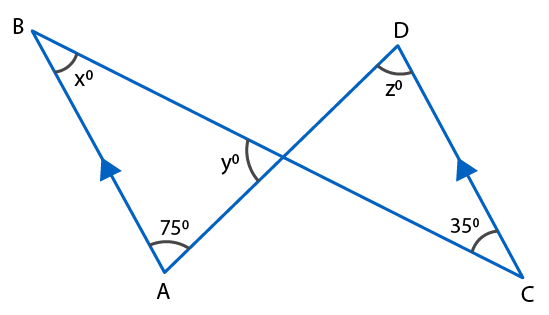Solution:

It is given that AB || CD and BC is a transversal

From the figure we know that ∠ABC = ∠BCD

So we get

x = 35

It is also given that AB || CD and AD is a transversal

So we get

z = 75

According to the △ ABO

We can write

∠ABO + ∠BAO + ∠BOA = 180o

By substituting the values

xo + 75o + yo = 180o

35o + 75o + yo = 180o

On further calculation

yo = 180o – 35o – 75o

By subtraction

yo = 180o – 110o

yo = 70o

Therefore, the value of x, y and z is 35, 70 and 75.

15. In the given figure, AB || CD. Prove that ∠BAE – ∠ECD = ∠AEC.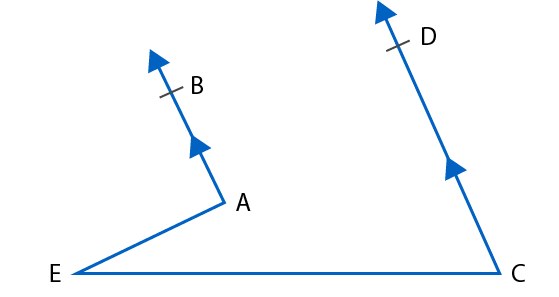Solution:

Construction a line EF which is parallel to AB and CD through the point E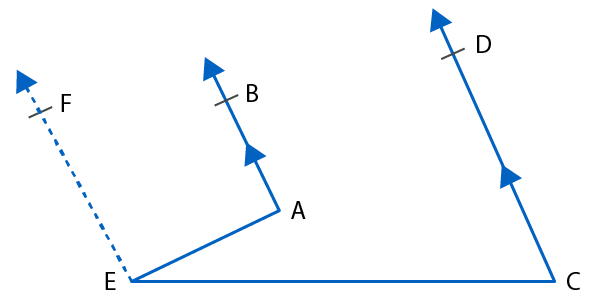We know that EF || AB and AE is a transversal

From the figure we know that ∠BAE and ∠AEF are supplementary

So it can be written as

∠BAE + ∠AEF = 180o….. (1)

We also know that EF || CD and CE is a transversal

From the figure we know that ∠DCE and ∠CEF are supplementary

So it can be written as

∠DCE + ∠CEF = 180o

According to the diagram the above equation can be written as

∠DCE + (∠AEC +∠AEF) = 180o

From equation (1) we know that ∠AEF can be written as 180o – ∠BAE

So we get

∠DCE + ∠AEC + 180o – ∠BAE = 180o

So we get

∠BAE – ∠DCE = ∠AEC

Therefore, it is proved that ∠BAE – ∠DCE = ∠AEC

16. In the given figure, AB || CD. Prove that p + q – r = 180.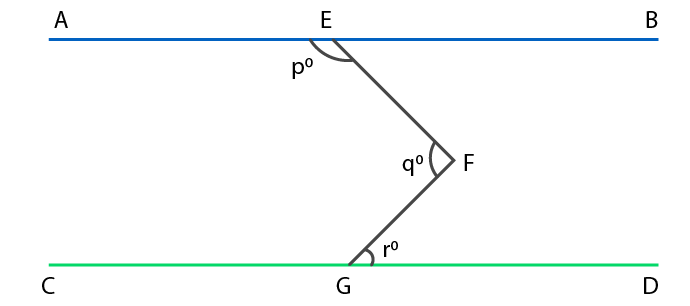Solution:

Draw a line KH passing through the point F which is parallel to both AB and CD

We know that KF || CD and FG is a transversal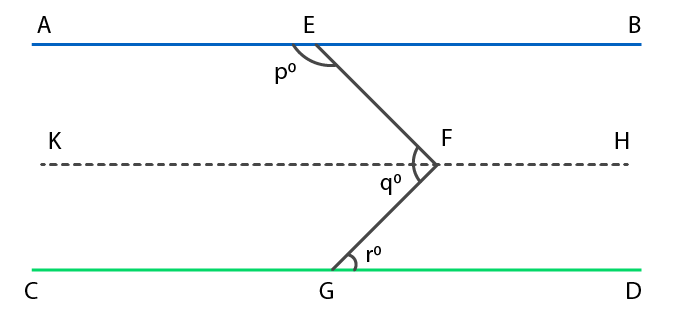From the figure we know that ∠KFG and ∠FGD are alternate angles

So we get

∠KFG = ∠FGD = ro ……. (1)

We also know that AE || KF and EF is a transversal

From the figure we know that ∠AEF and ∠KFE are alternate angles

So we get

∠AEF + ∠KFE = 180o

By substituting the values we get

po + ∠KFE = 180o

So we get

∠KFE = 180o – po ……. (2)

By adding both the equations (1) and (2) we get

∠KFG + ∠KFE = 180o – po + ro

From the figure ∠KFG + ∠KFE can be written as ∠EFG

∠EFG = 180o – po + ro

We know that ∠EFG = qo

qo = 180o – po + ro

It can be written as

p + q – r = 180o

Therefore, it is proved that p + q – r = 180o

17. In the given figure, AB || CD and EF || GH. Find the values of x, y, z and t.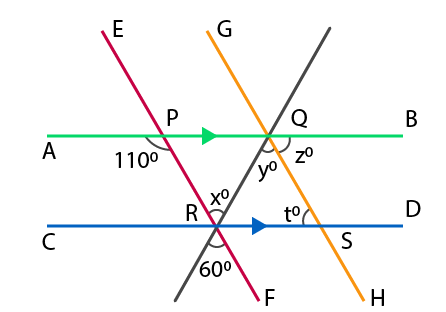Solution:

From the figure we know that ∠PRQ = xo = 60o as the vertically opposite angles are equal

We know that EF || GH and RQ is a transversal

From the figure we also know that ∠PRQ and ∠RQS are alternate angles

So we get

∠PRQ = ∠RQS

∠x = ∠y = 60o

We know that AB || CD and PR is a transversal

From the figure we know that ∠PRD and ∠APR are alternate angles

So we get

∠PRD = ∠APR

It can be written as

∠PRQ + ∠QRD = ∠APR

By substituting the values we get

x + ∠QRD = 110o

60o + ∠QRD = 110o

On further calculation

∠QRD = 110o – 60o

By subtraction

∠QRD = 50o

According to the △ QRS

We can write

∠QRD + ∠QSR + ∠RQS = 180o

By substituting the values

∠QRD + to + yo = 180o

50o + to + 60o = 180o

On further calculation

to = 180o – 50o – 60o

By subtraction

to = 180o – 110o

to = 70o

We know that AB || CD and GH is a transversal

From the figure we know that zo and to are alternate angles

So we get

zo = to = 70o

Therefore, the values of x, y, z and t are 60o, 60o, 70o and 70o.

18. In the given figure, AB || CD and a transversal t cuts them at E and F respectively. If EG and FG are the bisectors of ∠BEF and ∠EFD respectively, prove that ∠EGF = 90o.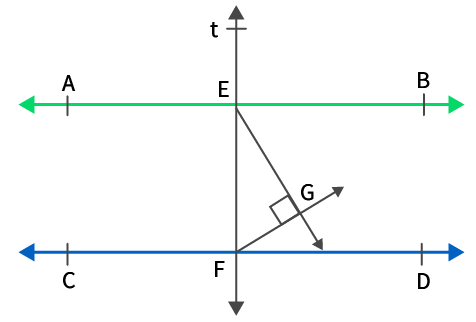Solution:

We know that AB || CD and t is a transversal cutting at points E and F

From the figure we know that ∠BEF and ∠DFE are interior angles

So we get

∠BEF + ∠DFE = 180o

Dividing the entire equation by 2 we get

(1/2)∠BEF + (1/2) ∠DFE = 90o

According to the figure the above equation can further be written as

∠GEF + ∠GFE = 90o ……. (1)

According to the △ GEF

We can write

∠GEF + ∠GFE + ∠EGF = 180o

Based on equation (1) we get

90o + ∠EGF = 180o

On further calculation

∠EGF = 180o – 90o

By subtraction

∠EGF = 90o

Therefore, it is proved that ∠EGF = 90o

19. In the given figure, AB || CD and a transversal t cuts them at E and F respectively. If EP and FQ are the bisectors of ∠AEF and ∠EFD respectively, prove that EP || FQ.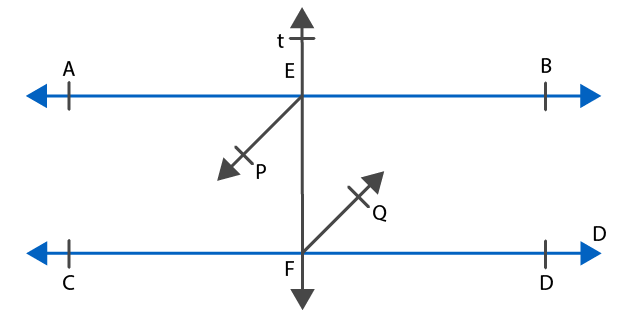Solution:

We know that AB || CD and t is a transversal

From the figure we know that ∠AEF and ∠EFD are alternate angles

So we get

∠AEF = ∠EFD

Dividing both the sides by 2 we get

(1/2) ∠AEF = (1/2) ∠EFD

So we get

∠PEF = ∠EFQ

The alternate interior angles are formed only when the transversal EF cuts both FQ and EP.

Therefore, it is proved that EP || FQ.

20. In the given figure, BA || ED and BC || EF. Show that ∠ABC = ∠DEF.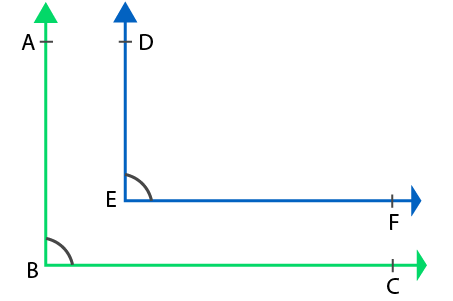Solution:

Extend the line DE to meet the line BC at the point Z.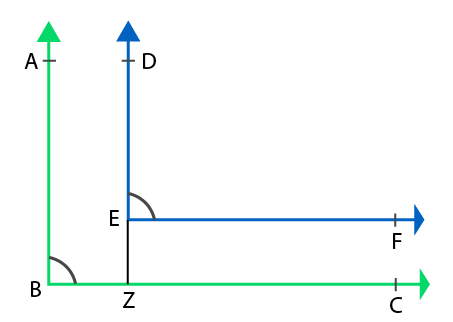We know that AB || DZ and BC is a transversal

From the figure we know that ∠ABC and ∠DZC are corresponding angles

So we get

∠ABC = ∠DZC ….. (1)

We also know that EF || BC and DZ is a transversal

From the figure we know that ∠DZC and ∠DEF are corresponding angles

So we get

∠DZC = ∠DEF …… (2)

Considering both the equation (1) and (2) we get

∠ABC = ∠DEF

Therefore, it is proved that ∠ABC = ∠DEF

21. In the given figure, BA || ED and BC || EF. Show that ∠ABC + ∠DEF = 180o.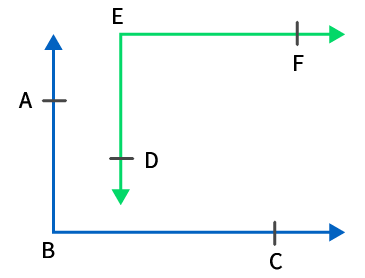Solution:

Extend the line ED to meet the line BC at the point Z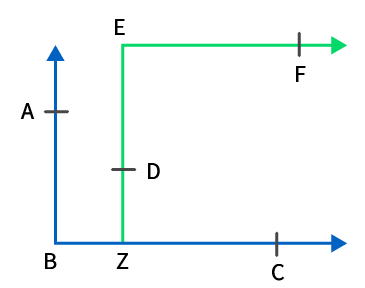We know that AB || EZ and BC is a transversal

From the figure we know that ∠ABZ and ∠EZB are interior angles

So we get

∠ABZ + ∠EZB = 180o

∠ABZ can also be written as ∠ABC

∠ABC + ∠EZB = 180o …… (1)

We know that EF || BC and EZ is a transversal

From the figure we know that ∠BZE and ∠ZEF are alternate angles

So we get

∠BZE = ∠ZEF

∠ZEF can also be written as ∠DEF

∠BZE = ∠DEF ….. (2)

By substituting equation (1) in (2) we get

∠ABC + ∠DEF = 180o

Therefore, it is proved that ∠ABC + ∠DEF = 180o

22. In the given figure, m and n are two plane mirrors perpendicular to each other. Show that the incident ray CA is parallel to the reflected ray BD.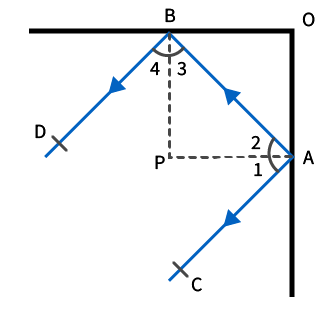Solution:

Construct a line m and n from A and B intersect at P

So we get

OB ⊥ m and OC ⊥ n

So m ⊥ n

We can also write it as

OB ⊥ OC

Since APB is a right angle triangle

We know that ∠APB = 90o

So we can write it as

∠APB = ∠PAB + ∠PBA

By substituting the values

90o = ∠2 + ∠3

We know that angle of incidence is equal to the angle of reflection

So we get

∠1 = ∠2 and ∠4 = ∠3

It can be written as

∠1 + ∠4 = ∠2 + ∠3 = 90o

We can write it as

∠1 + ∠2 + ∠3 + ∠4 = 180o

We know that ∠1 + ∠2 = ∠CAB and ∠3 + ∠4 = ∠ABD

∠CAB + ∠ABD = 180o

According to the diagram ∠CAB and ∠ABD are consecutive interior angles when the transversal AB cuts BD and CA.

Therefore, it is proved that CA || BD.

23. In the figure given below, state which lines are parallel and why?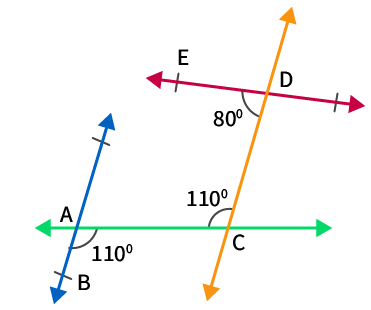Solution:

According to the figure we know that ∠BAC and ∠ACD are alternate angles

So we get

∠BAC = ∠ACD = 110o

We know that ∠BAC and ∠ACD are alternate angles when the transversal AC cuts CD and AB.

Therefore, AB || CD.

24. Two lines are respectively perpendicular to two parallel lines. Show that they are parallel to each other.

Solution: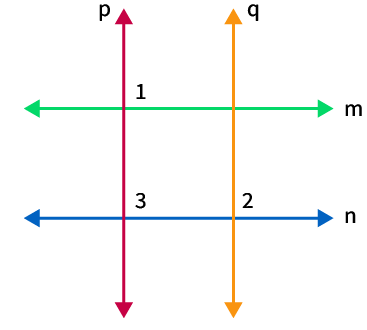According to the figure consider the two parallel lines as m and n

We know that p ⊥ m and q ⊥ n

So we get

∠1 = ∠2 = 90o

We know that m || n and p is a transversal

From the figure we know that ∠1 and ∠3 are corresponding angles

So we get

∠1 = ∠3

We also know that

∠2 = ∠3 = 90o

We know that ∠2 and ∠3 are corresponding angles when the transversal n cuts p and q.

So we get p || q.

Therefore, it is shown that the two lines which are perpendicular to two parallel lines are parallel to each other.

### RS Aggarwal Solutions for Class 9 Maths Chapter 7: Lines and Angles

Chapter 7, Lines and Angles, has 3 exercises from RS Aggarwal Solutions textbook, which covers entire topics to make the students ready to face the exams with higher confidence. Major topics which are explained in brief under this Chapter are as given below:

• Basic Terms and Definitions
• Various types of Angles
• Some Angle Relations
• Some Results on Angle Relations
• Results on Parallel Lines
• Corresponding Angles Axiom

### Also access RS Aggarwal Solutions Class 9 Maths Chapter 7 – Lines and Angles Exercises

Exercise 7A Solutions 12 Questions

Exercise 7B Solutions 16 Questions

Exercise 7C Solutions 24 Questions

### Chapter brief of RS Aggarwal Solutions Class 9 Maths Chapter 7 – Lines and Angles

For a better conceptual knowledge, the students can make use of RS Aggarwal Solutions for Class 9 as a reference material to perform well in the exams. The step by step description of the solutions in a simple language is done by our faculty, based on the understanding ability of the students.

Lines and Angles have a wide range of applications in various fields like designing of roads, buildings, sports facilities and lines are mainly used to plan the line of business, which gives information about the services and products offered by the company. The students can download the PDF format of the solutions attached here in order to gain a better conceptual knowledge for the board exams.